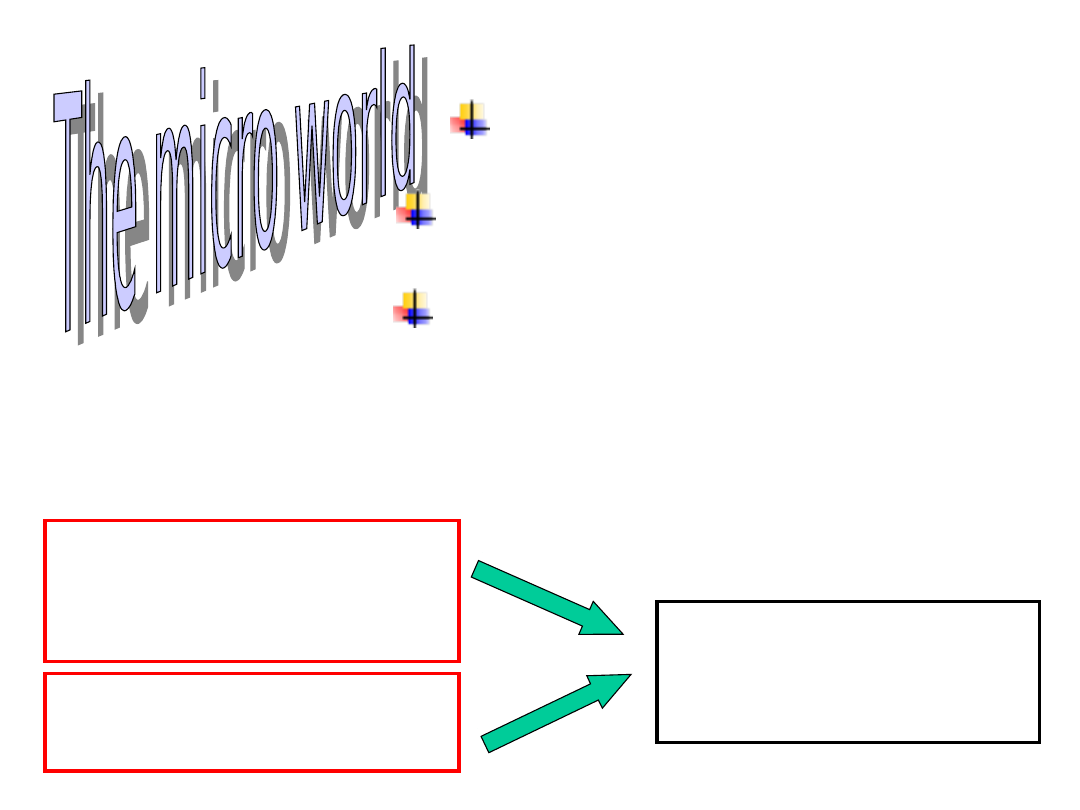Diffusion

Thermal fluctuations

Low Reynold’s number

nm.

the range 2 - 10 nm.

Fluid can be

considered as a

continuum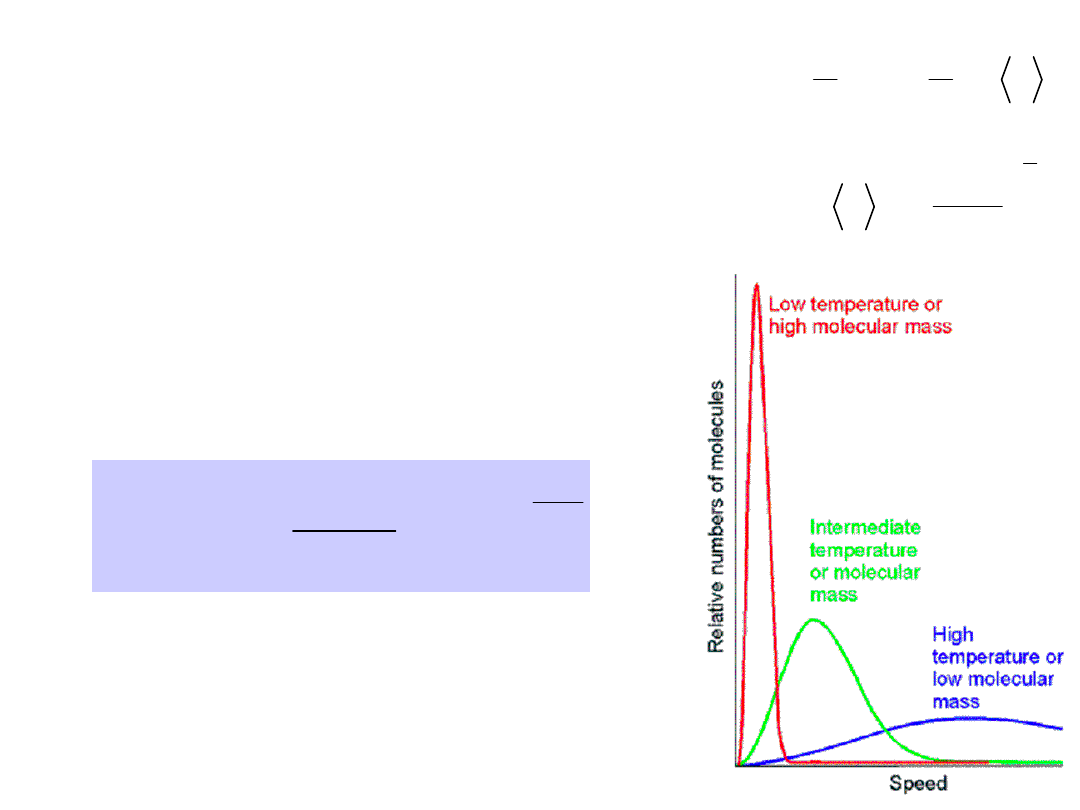The distribution of molecular

speeds with temperature and

molar mass

Actual velocity  Maxwell’s distribution

RT

Mv

e

v

RT

M

v

f

2

2

2

/

3

2

2

4

)

(

Molecular speed

2

1

2

3

2

1

2

3

M

kT

v

v

M

kT

For T = 300 K

500 Da (ATP) – v = 70 m/s

50 000 Da (protein) – v = 7 m/s

6.25 GDa (200 nm diameter vesicle) –
v = 600 μm/s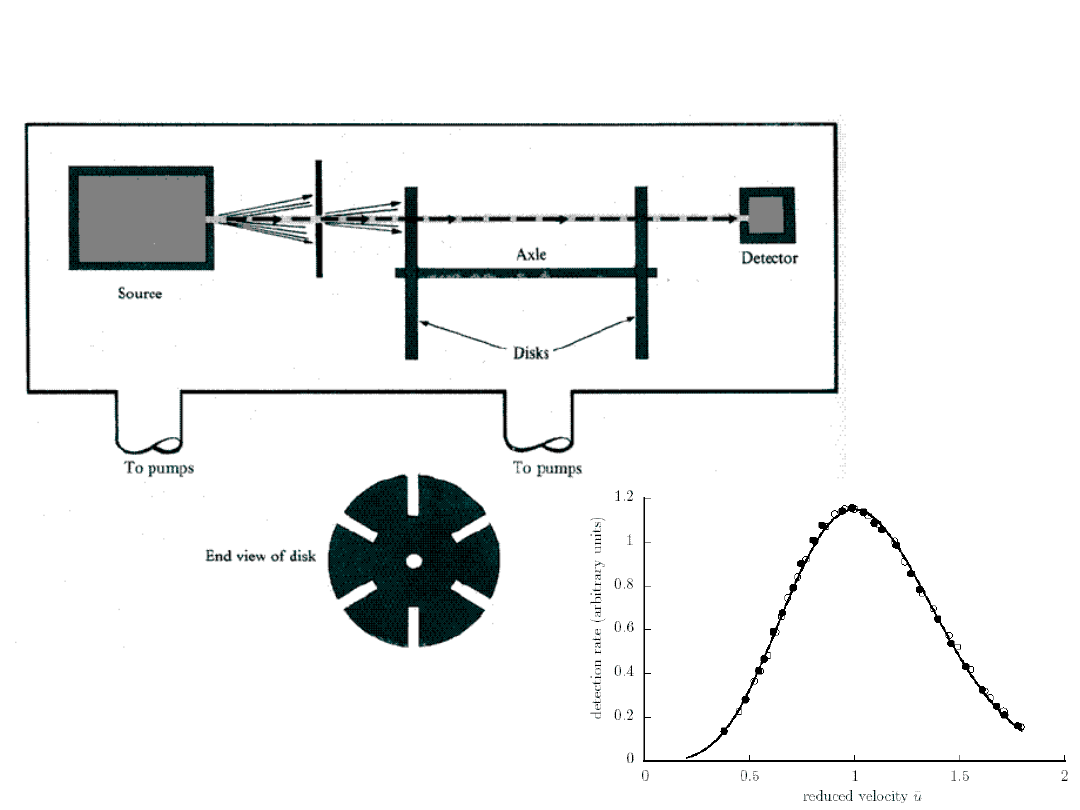An experimental apparatus to

measure the distribution of

molecular speeds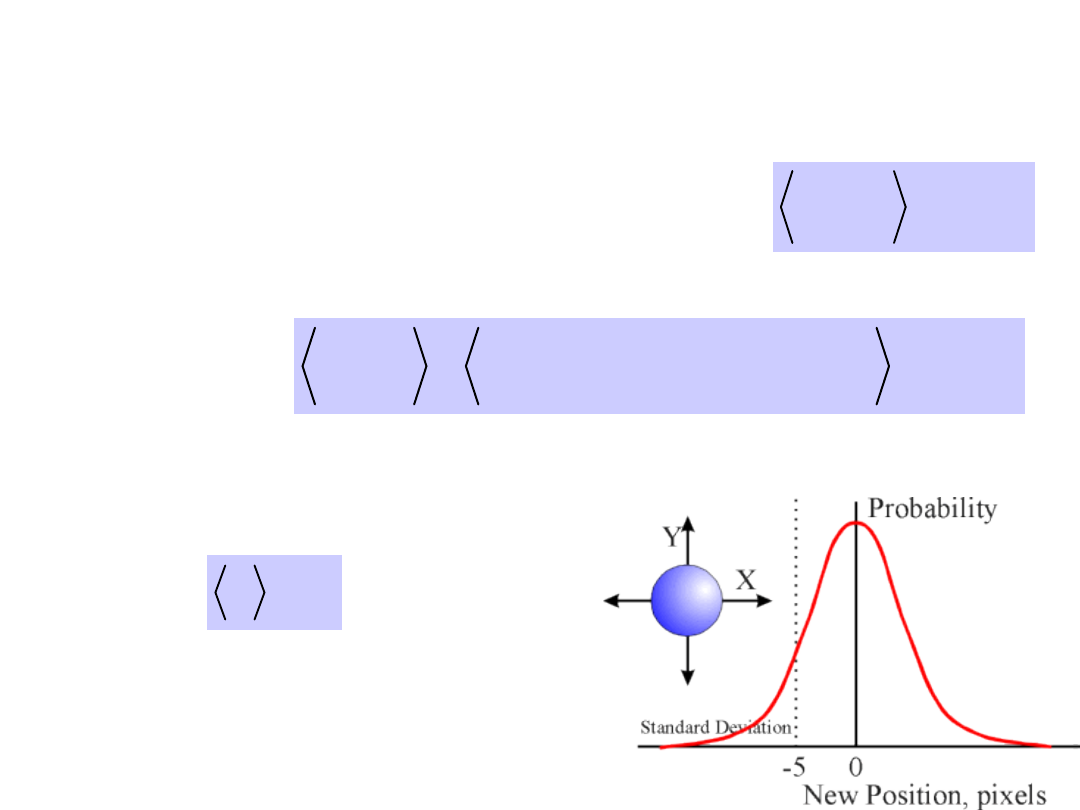The mean-square dosplacement in

a one-dimensional random walk.

 

Dt

x

N

2

2

 

     

Dt

z

y

x

r

N

N

N

N

6

2

2

2

2

3D diffusion.

No net movement

occurs.

0

x

The distribution is symmetrical.

Diffusion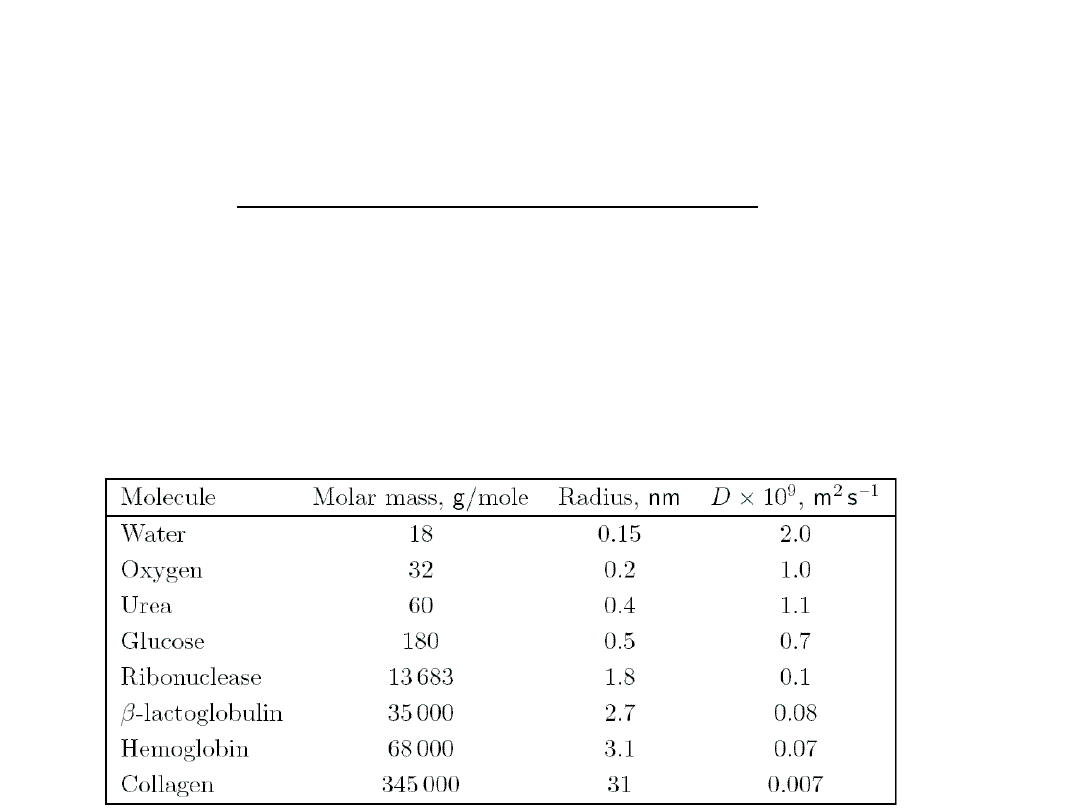Net solute movement is from higher to lower

concentration,

even

though

individual

particles move completely randomly.

The non-uniform distribution provides an

energy gradient to drive the overall process of
net movement from high to low concentration.

In thermodynamic terms, we're watching the

increase in entropy within a small, isolated

system without an input of energy.

Diffusion of selected molecules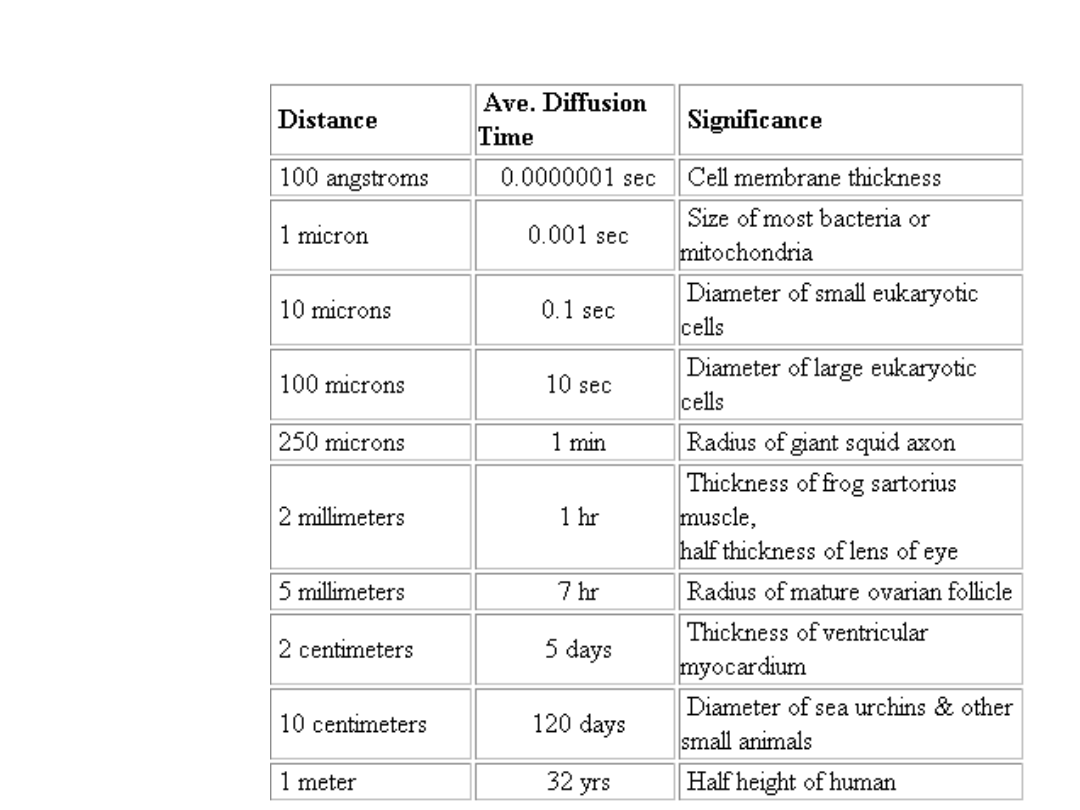D

10

-5

for most small molecules in water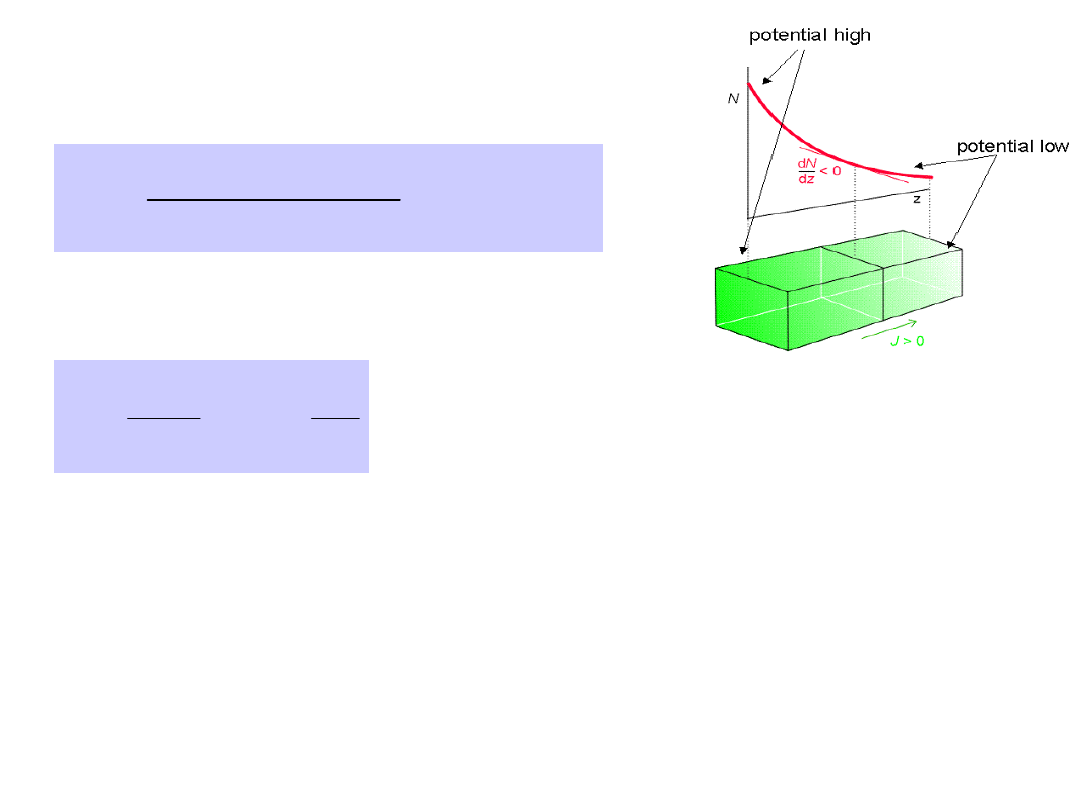Force

dx

potential

d

J

)

(

More probability seems to be „pushing” the

particles.

The random walk results with deterministic flow of particles.

But only when number of particles is large.

Flux of particles due to diffusion

dx

dc

D

dm

j

Fick’s first low

D – Diffusion

constant (m

2

/s)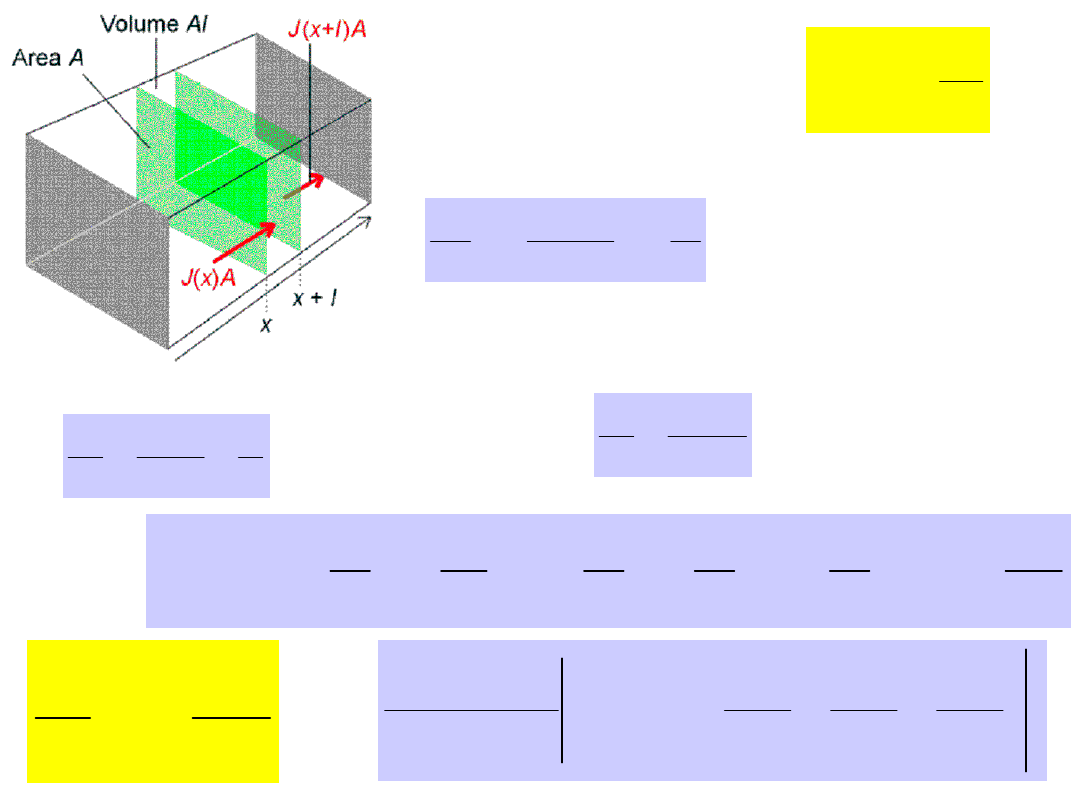Flux into volume A

l

l

J

Aldt

t

c

Flux out of volume A

l

l

J

Aldt

J

t

c

'

'

'

Flux through volume A

l

l

J

J

t

c

'

2

2

'

'

x

c

Dl

l

x

c

c

x

D

x

c

D

x

c

D

x

c

D

J

J

Fick’s 2

nd

law

2

2

x

c

D

t

c

x

c

D

J

t

z

y

x

dz

c

d

dy

c

d

dx

c

d

D

dt

z

y

x

dc





2

2

2

2

2

2

,

,

)

,

,

(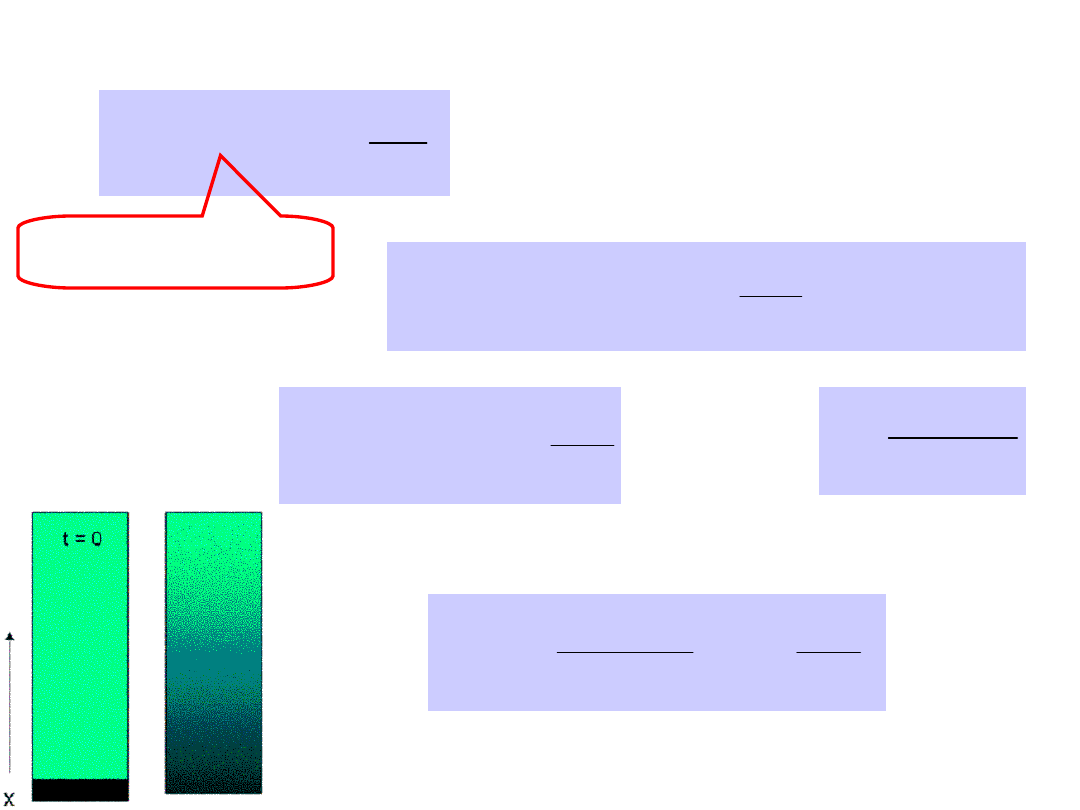Solution to diffusion equation





Dt

x

t

t

x

c

4

exp

)

,

(

2

2

/

1

Conditions:
At t = 0 all N

0

particles at x = 0

 

2

/

1

2

2

/

1

2

4

exp

D

dx

Dt

x

t

cdx

N









 

using



0

2

/

1

2

2

2

exp

r

dx

x

r

therefore

 

2

/

1

2 D

N





Dt

x

Dt

N

t

x

c

4

exp

2

)

,

(

2

2

/

1

In terms of particles per unit area

Strength of

source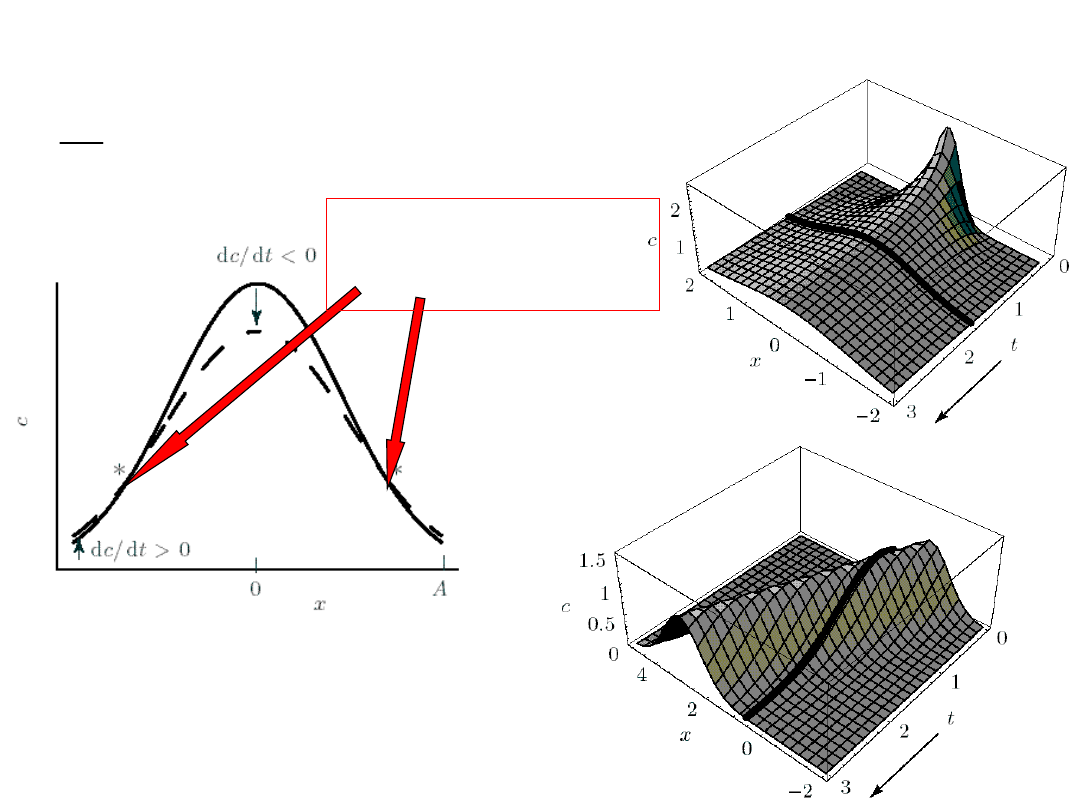Diffusion in space and time

The traveling wave

0

0

j

dt

dc

Inflection point

the curvature

changes signThermal conductiity

dz

dT

energy

J

)

(

dz

dv

momentum

the

of

component

x

J

x

)

(

Viscosity

A charged molecules in an electric field

dz

dV

J

1

)

charge

(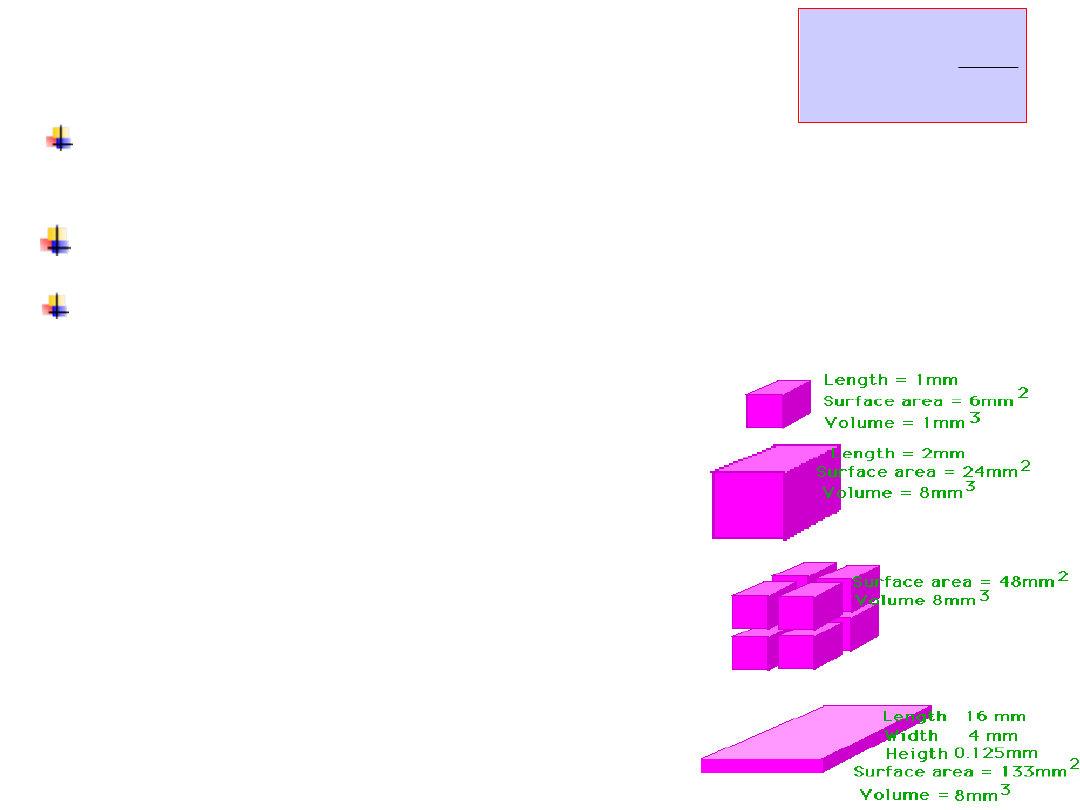Diffusive transport in biology

dx

dC

D

J

x

A concentration penalty

– diffusive

The time penalty

– diffusive transport

time scales as the square of the distance or
<X

2

> = 4Dt

No

directional

specificity

The size limit

As a cell gets bigger there

will come a time when its

surface area is insufficient

to meet the demands of the

cell's volume and the cell

stops growing or it will

divide.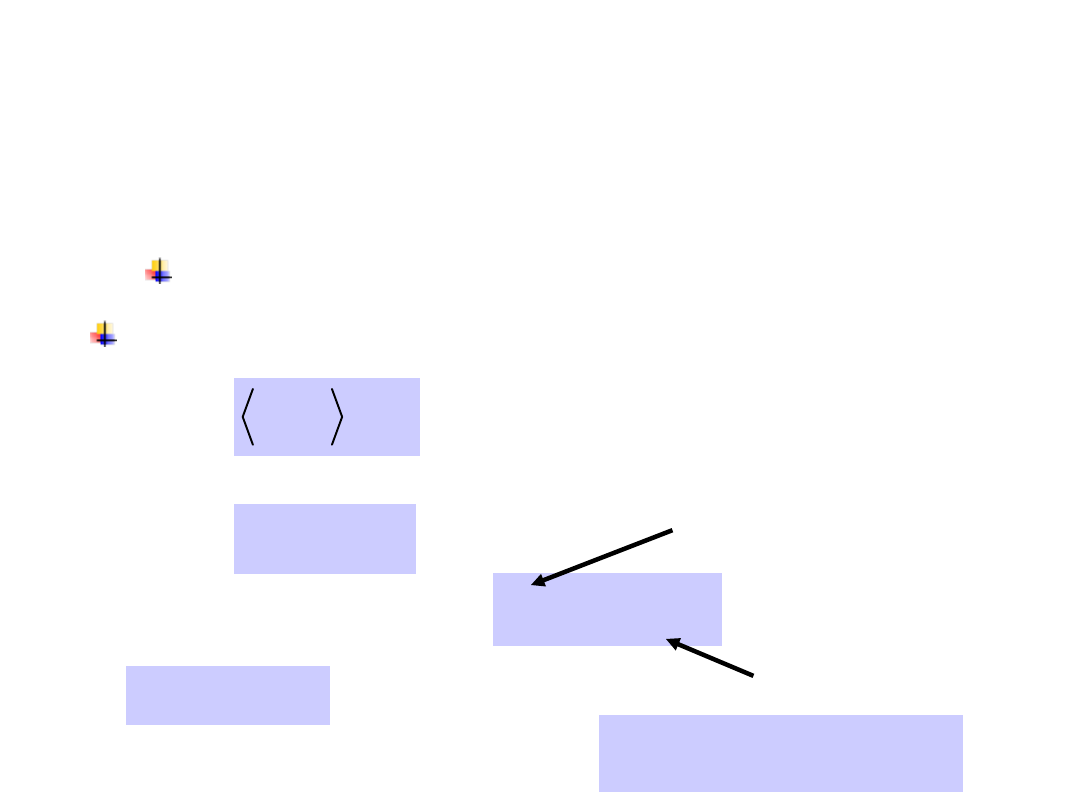Forces acting on a particle due to the
solvent:

(i)

Stochastic thermal (Langevin) force

:

Averageing over a large number of particles

The Langevin approach –

dissipative force

changes direction and magnitude

averages to zero over time

0

)

(

t

(ii)

a viscous drag force that always slows

the motions.

v

f

friction (damping) coeff.

viscosity

Stokes law

R



6

The thermal forces

nN

f

5

.

4

The gravitational force

f

nN

F

g



 14

10Newton’s law for the protein motion in a one-
dimensional domain of length L, x(t):

L

t

x

t

f

v

dt

dv

m

v

dt

dx

B

)

(

0

)

(

,

)

(

)

(

2

)

(

2

2

2

2

2

2

t

xf

dt

x

d

mv

dt

x

d

m

B

The average over a large number of proteins

)

(

2

2

2

2

2

2

2

t

xf

dt

x

d

mv

dt

x

d

m

B

The mass, m, of a typical protein is about 10

-21

kg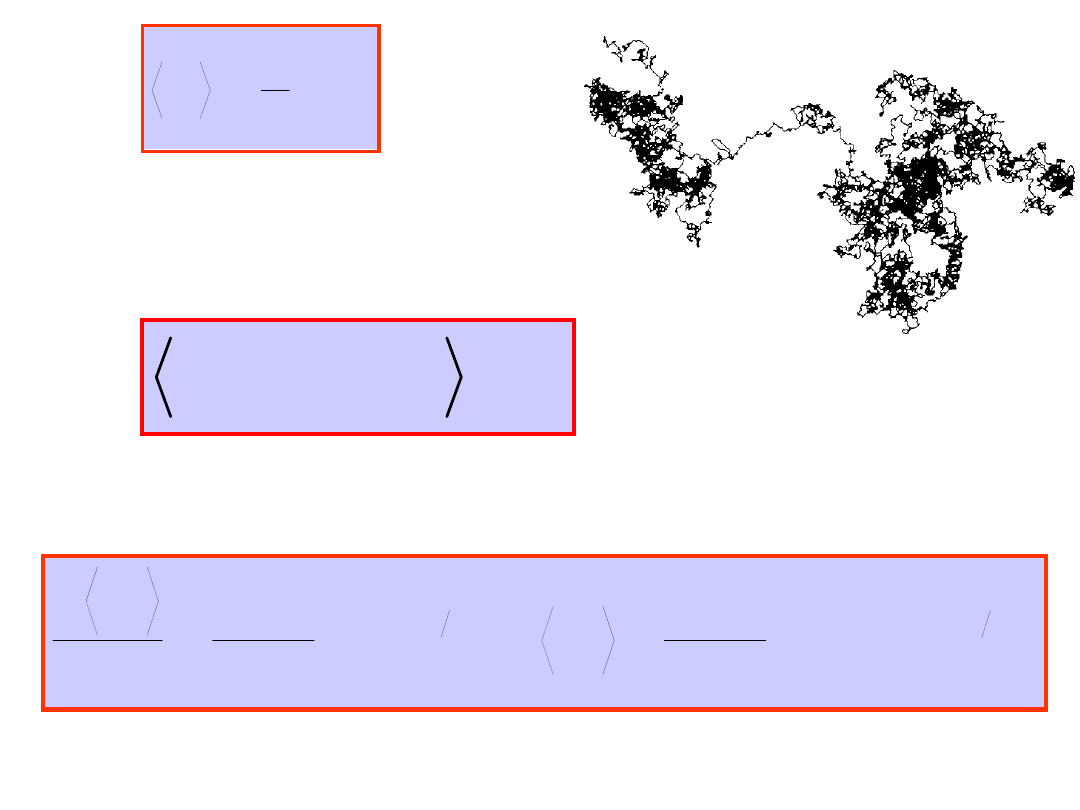Integrating twice between t = (0, t) with
x(0) = 0:

)

1

(

2

),

1

(

2

2

2

t

B

t

B

e

t

T

k

x

e

T

k

dt

x

d

where

 =

m/

.

T

k

E

B

2

1

The random impulses from

the water molecules are

uncorrelated with position.

0

)

(

)

(

t

f

t

x

B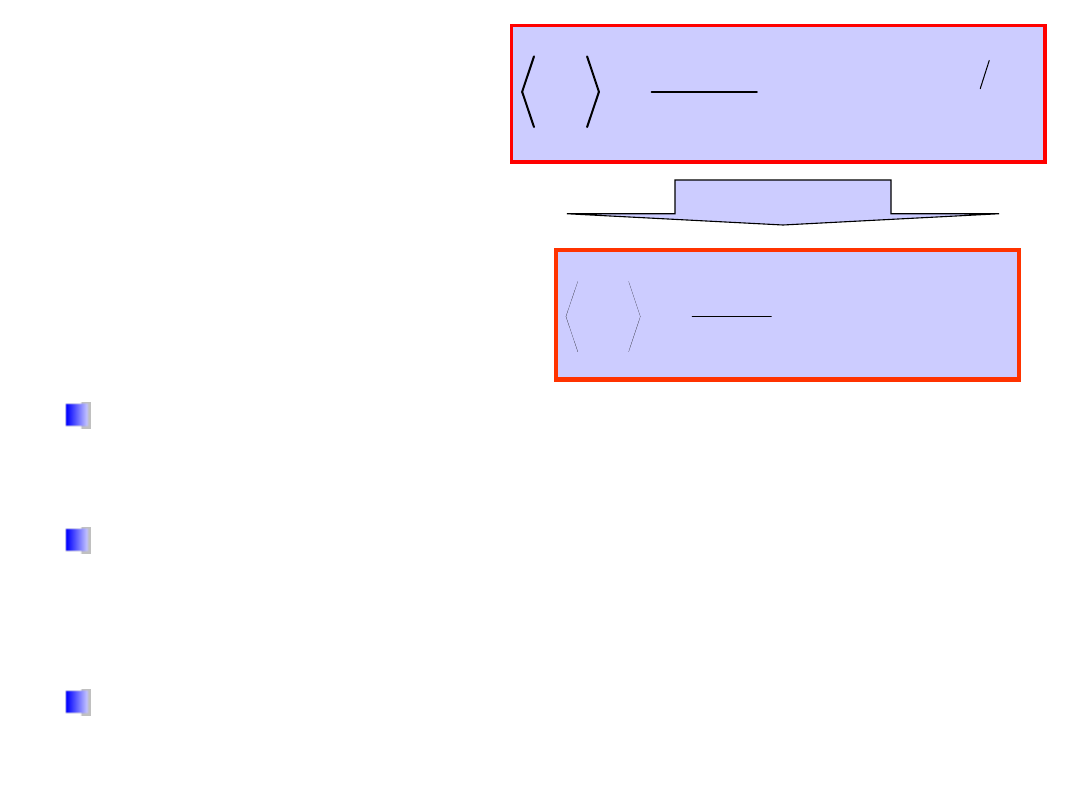The protein behaves as a ballistic particle moving

with a velocity

v = (k

B

T/m)

1/2

. For a protein with m

= 10

-21

kg,

v = 2 m/s.

In a fuid the protein moves at this velocity only

for a time

~ m/ = 10

-13

sec

– shorter than any

motion of interest in a molecular motor.

During this time the protein travels a distance

v

· ~ 0.01 nm

before it collides with another

molecule.

For short times,

t <<

,

the exponential can be
expand to second
order:

)

(

2

2



t

t

m

T

k

x

B

)

1

(

2

2

t

B

e

t

T

k

x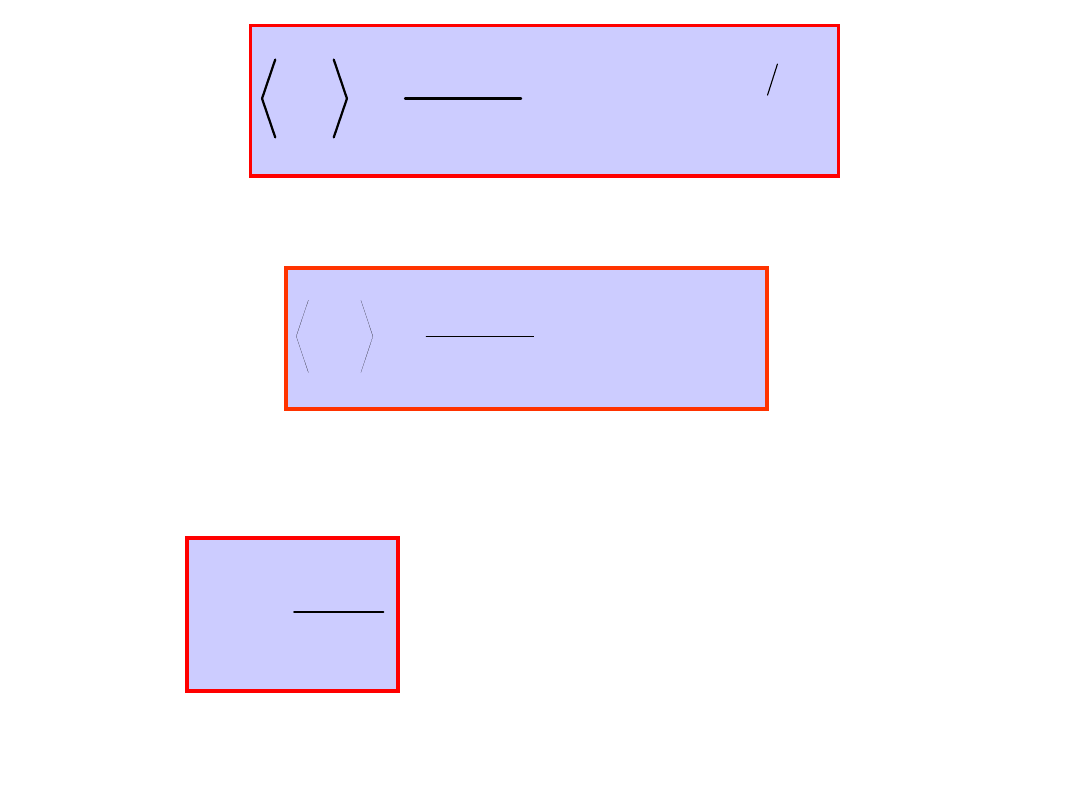)

(

2

2



t

t

T

k

x

B

When t >> , the exponential term disappears and:

For protein typically D ~ 10

-11

m

2

/sec.

Because

<x

2

> = 2Dt

(Einstein

relation – 1905):

T

k

D

B

Friction is

quantitatively related

to diffusion

)

1

(

2

2

t

B

e

t

T

k

xExternal forces acting on

macromolecules

)

(

)

,

(

t

f

t

t

x

dt

dx

B

)

(

)

,

(

t

f

t

t

x

dt

dx

B

Langevin equation

The inertial term
is neglected.

)

(

)

,

(

t

f

t

x

F

dt

dx

B

Forces acting on proteins can be characterized by
a potential

x

t

x

t

x

F

)

,

(

)

,

(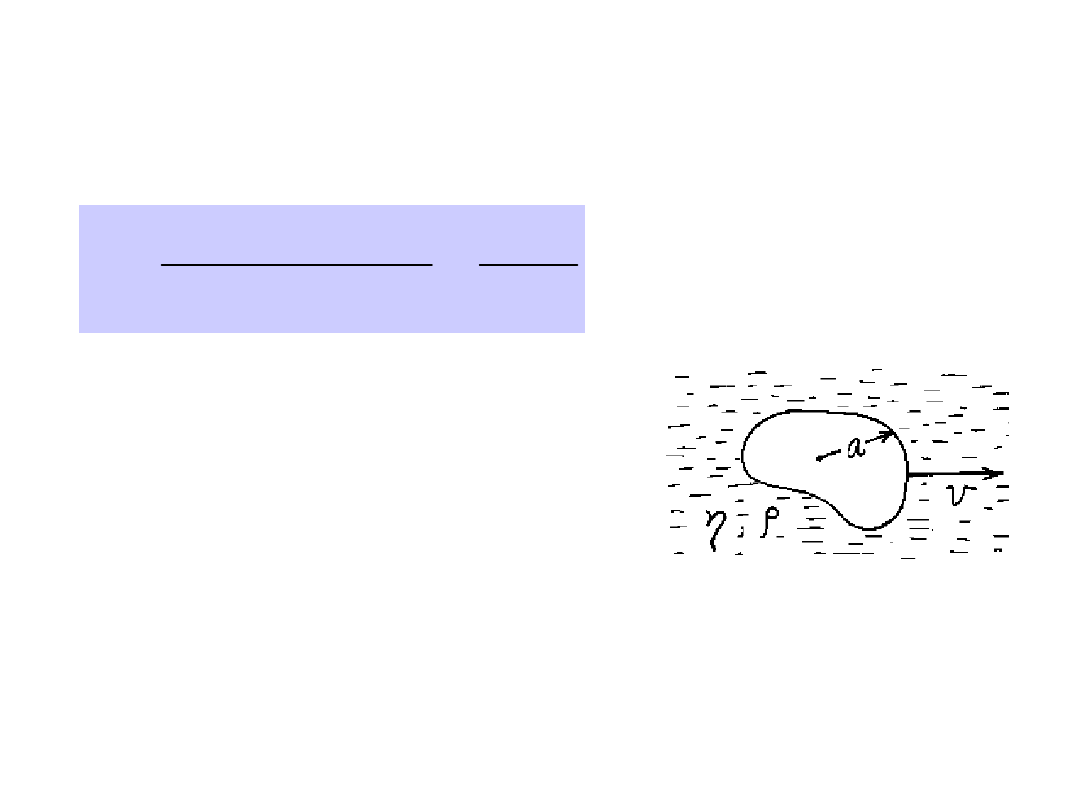It quantifies the relative importance of
friction and inertia

The Reynolds Number

The transition to turbulent flow in a pipe

occurs for R ~ 1000

Low Reynolds Number = Laminar

Flow

m

va

term

friction

term

inertial

R

a – radius of a particle

v – particle
velocity

m

– medium

density

When the Reynolds

number ‘R’ is small the

viscous forces dominate.Size spectrum of living organisms and

the biological and physical properties

associated with the scale.

10

-1

10

-1

1 min

10

-6

100 μm

Protozoan

10

3

5

1 day

1

1 cm

Shrimp

10

8

1000

10

3

years

10

9

10 m

Whale

10

-5

10

-3

1 msec

10

-12

1 μm

Bacterium

Reynol

ds

number

Swimmi

ng speed

[cm/s]

Diffusi

on time

Mass

[g]

Leng

th

10

5

20

1 week

10

2

10 cm

Herring

10

7

100

1 year

10

6

1 m

TunaWithout external force

such object will stop after

It takes it about 0.6 μsec

to stop.

Inertia plays no

role whatsoever !!

R ~ 10

–5

The objects which are the order of a μm in size is
moving in water with a typical speed of 30 μm/s.

(kg/m•sec at

20

o

C)

Water

10

-3

Olive oil

0.084

Glycerine

1.34

Glucose

10

13There are two solutions to the

problem of swimming at low

Reynolds number.

If the animal tries

to swim by a

reciprocal motion,

it can’t go
anywhere.

Fast or slow, it

exactly retraces its

trajectory and it’s

back where it

started.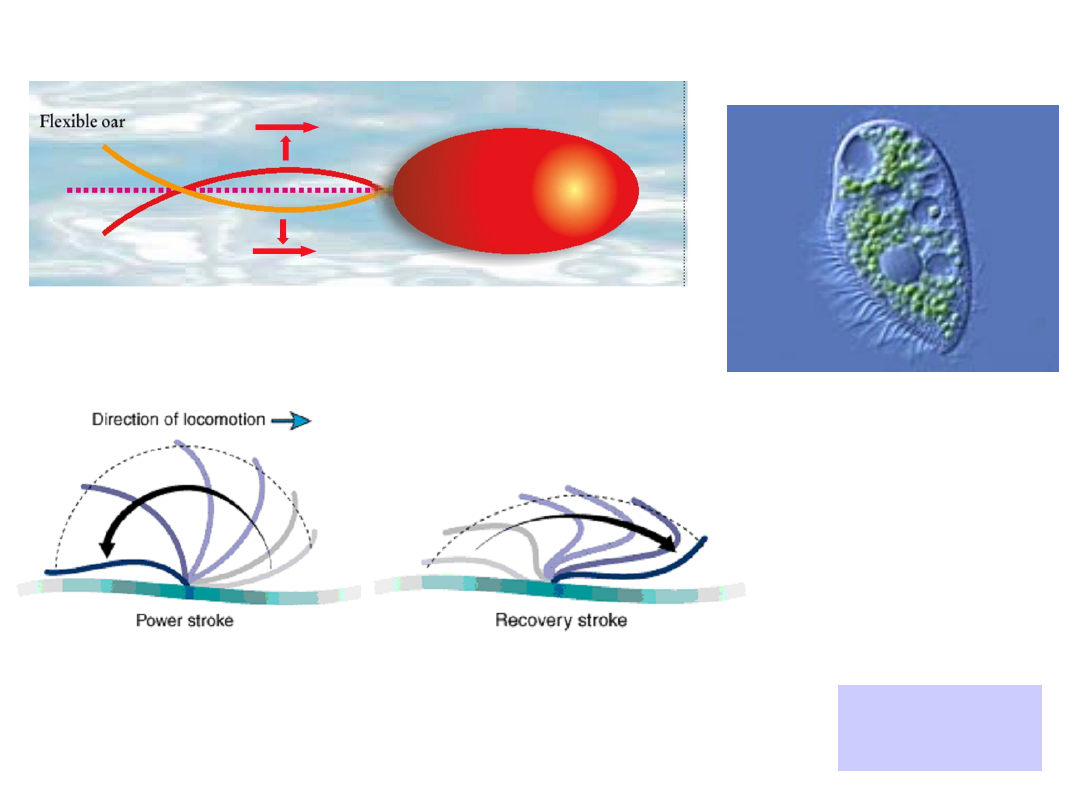coefficient can be anizotropic.

II

The flexible oar

The other possibility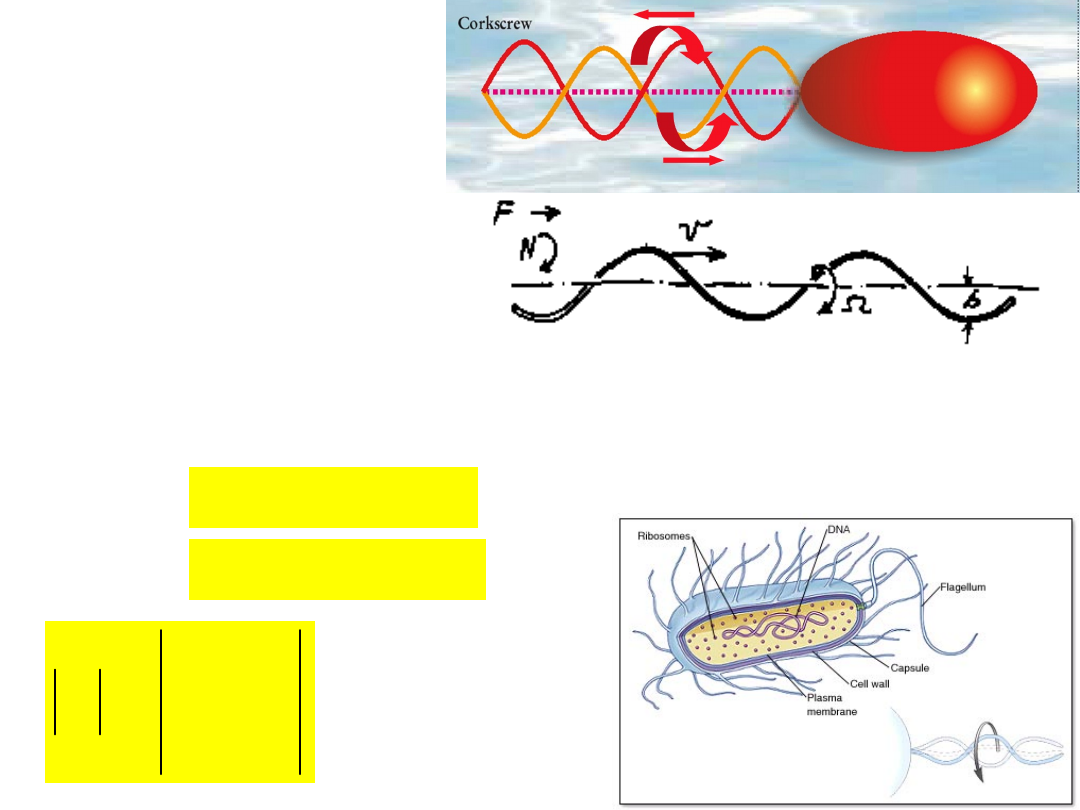E.
coli

Rotary motion is

periodic but not

reciprocal.

The helix can translate and it
can rotate.

D

C

B

A

P

The

propulsio

n matrix

At low Reynolds number everything is linear.

B

Av

F

Force

D

Cv

N

Torgue

v – velocity, Ω –angular velocity

A „corkscrew”

(flagella)

It is a rigid, helical object.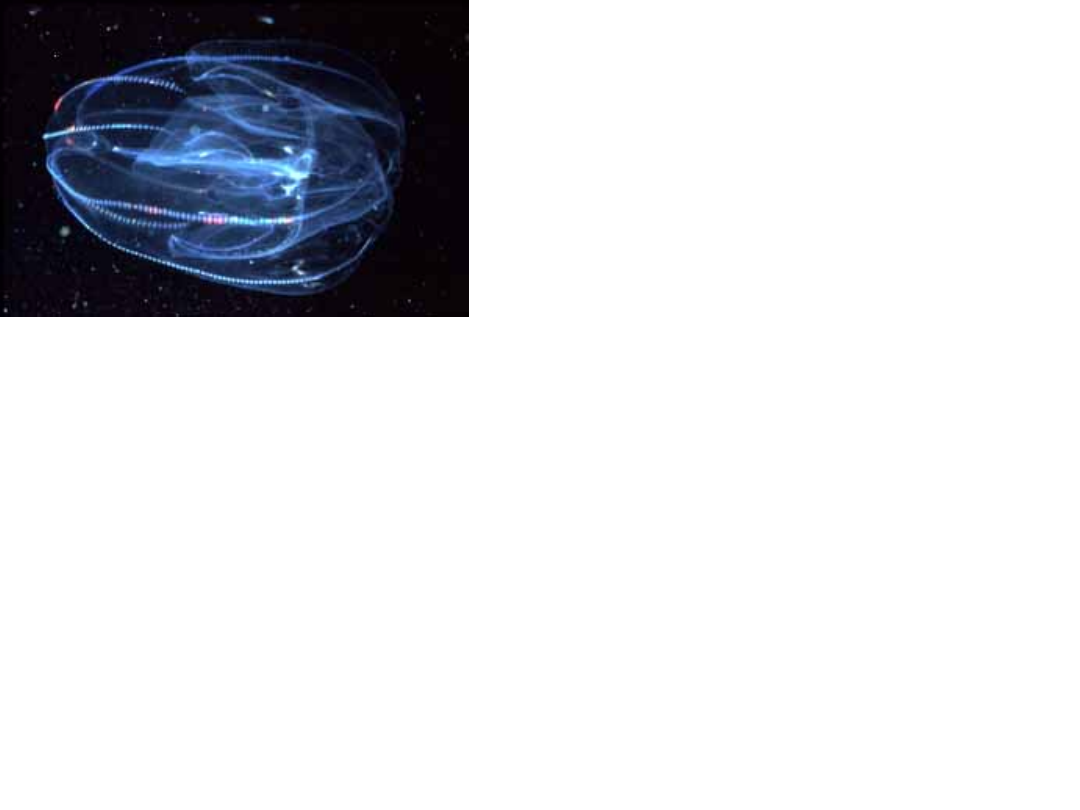At low Reynolds

number you can't shake

If you move, you take it

falls behind.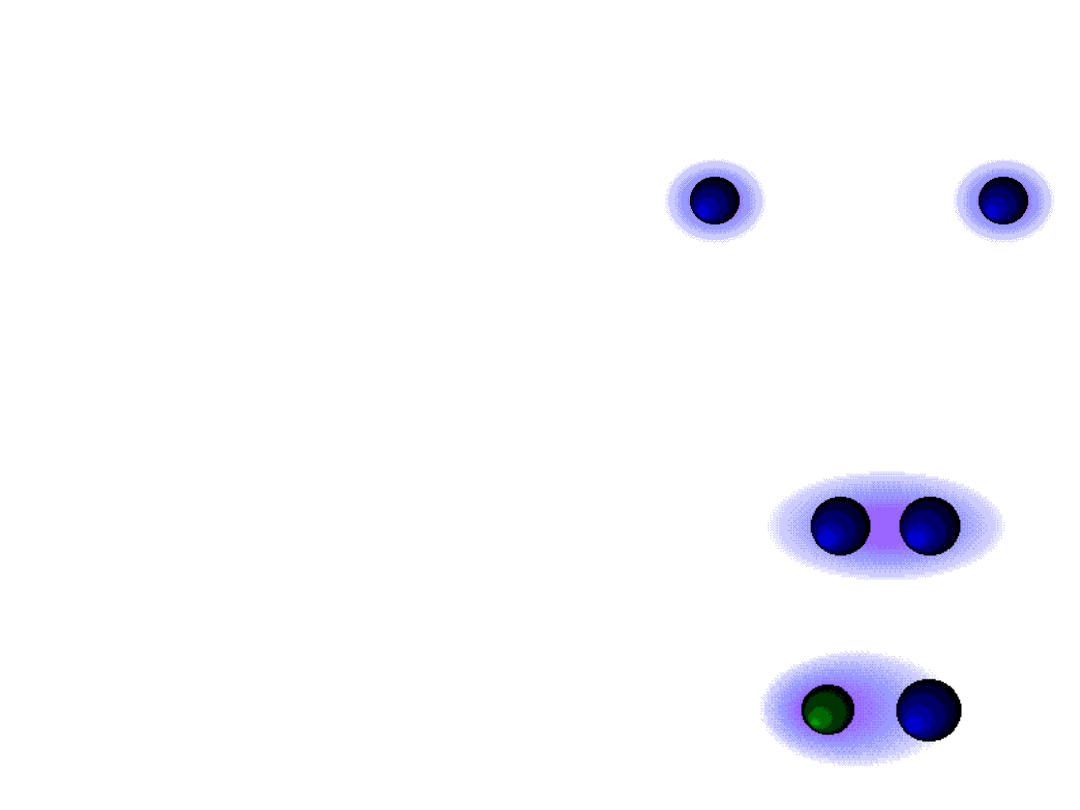A covalent bond

is formed between the two non-

metals which share a pair of valance electrons so
that each obtains a filled valence shell.

A non-polar covalent bond

the electrons are shared equally
between the two atoms.

A polar covalent bond

the

electrons are shared unequally
between the two atoms.

Bio-polymers are held together

by covalent bonds between

subunits.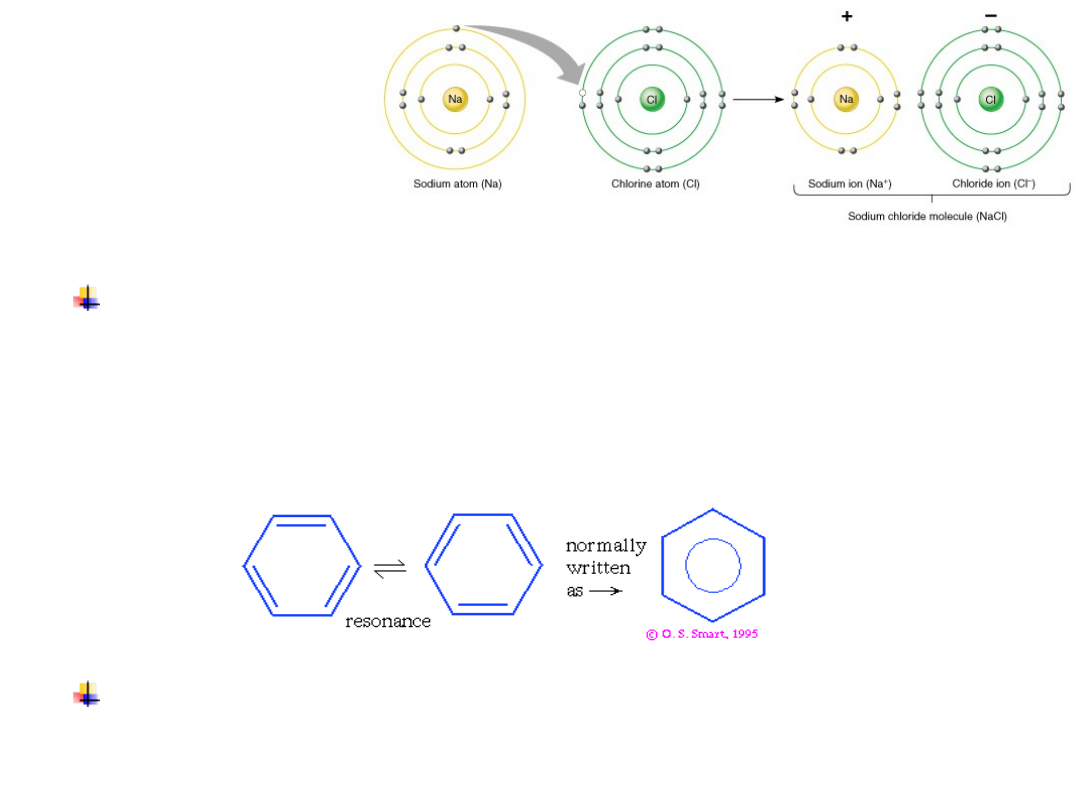Ionic Bonds

The valence electrons interact and the metal

transfers its valence electrons to the non-metal.

Delocaltzed bonding

– a resonance hybrid

between alternate structures e.g., benzene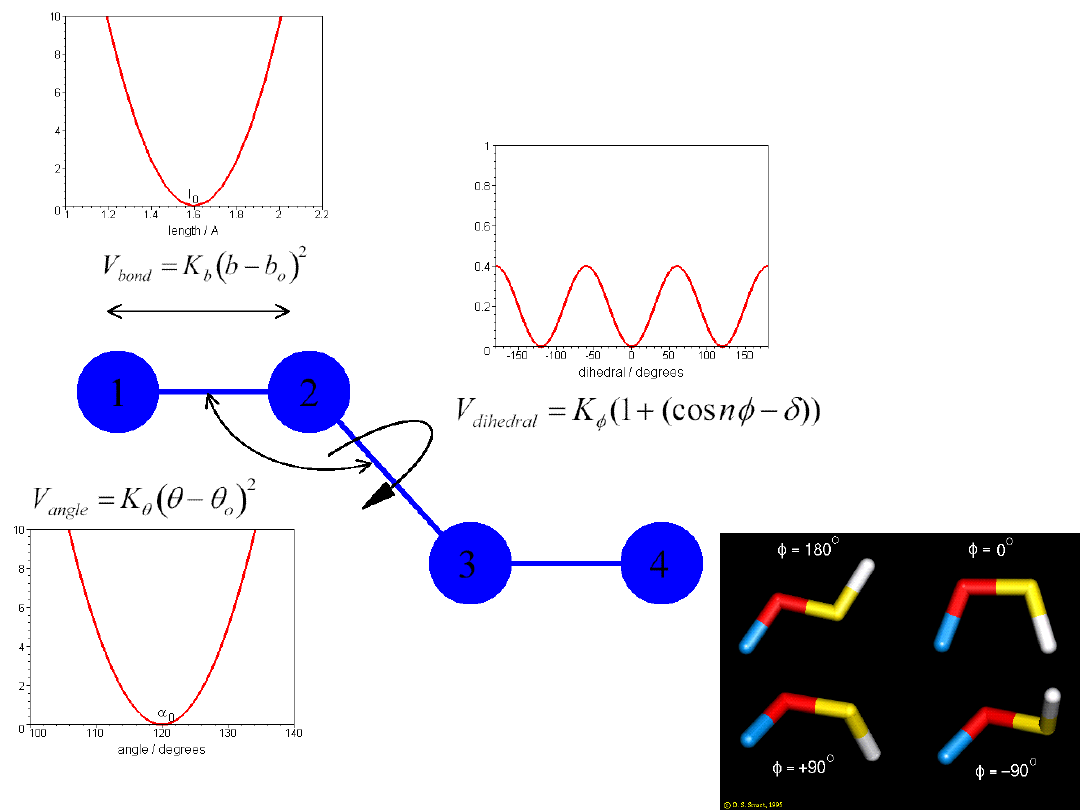Bond

energy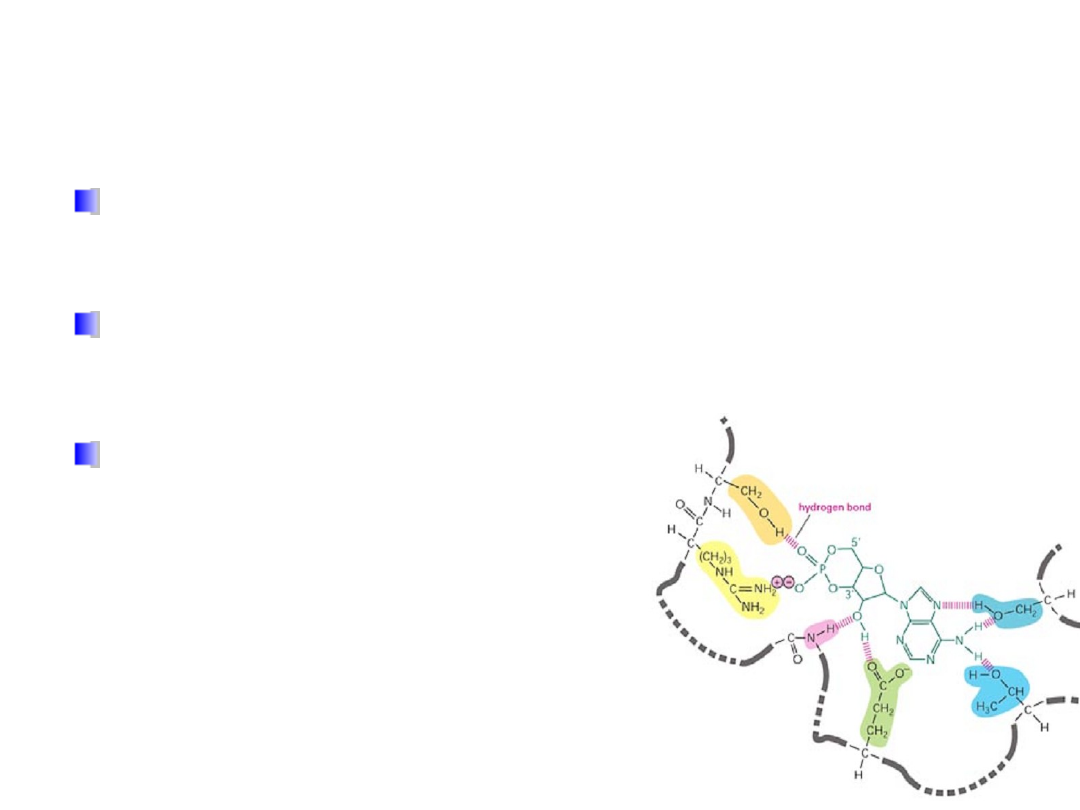The macromolecular structures have extremely

many degrees of conformational freedom.

Intra- and Inter-molecular

interactions is what biology

Every residue of a macromolecule, and every

bit of their surfaces, are interacting with their
surroundings.

These interactions are

what set the stability criteria
and the dynamic constraints
of the biological structures.

All that allow for dynamic interactions

(

Interactions form, break, re-form constantly)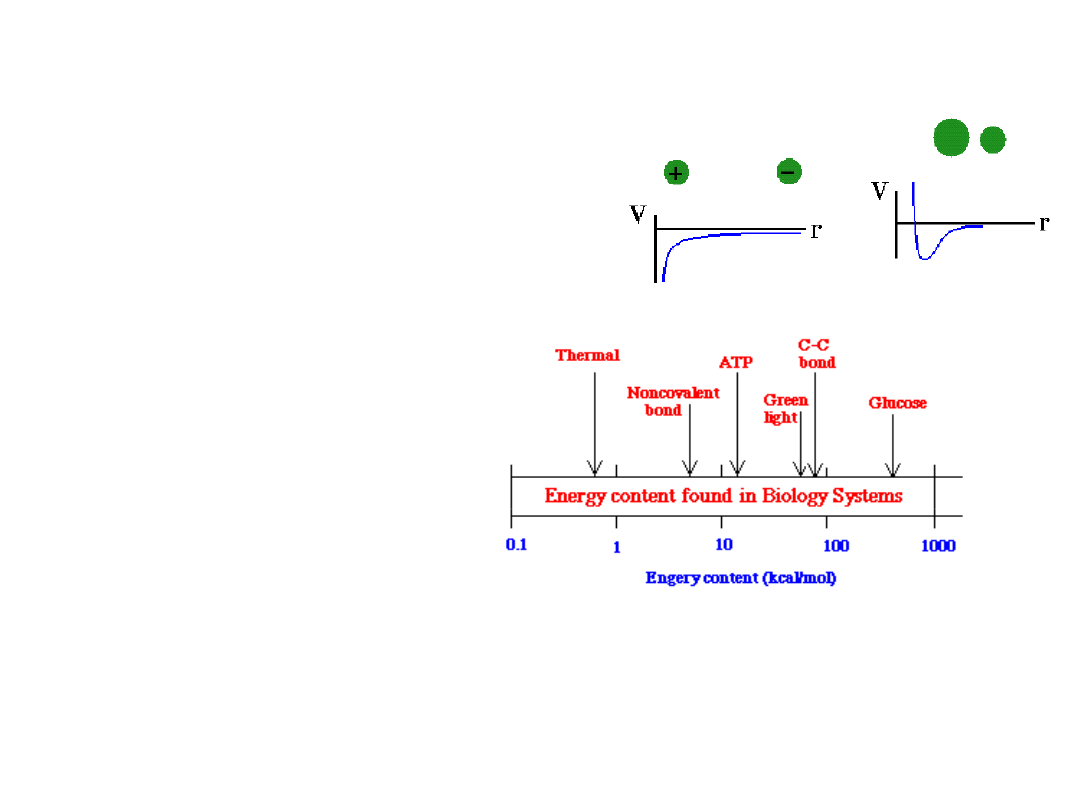steric

repulsion

Noncovalent interactions

hydrogen bonds

Noncovalent Interactions
are Weak

They are ~ 10-100 times weaker than covalent bonds
(< 10 kJ/mol).

Noncovalent interactions

essentially hold together the entire

organism.

electrostatics

van der Waals (repulsive and attractive)Interaction

Energy [kJ/mol]

Ion-ion

56 (ε = 8, r = 3Å)

Ion-dipol

–8 to +8 (μ = 2 debye)

Dipole-dipole

–2 to +2

Ion-induce dipole

0.24 (α = 10

-24

cm

3

)

Dispersion, and stacking

of aromatic ring system

0 to 40

H-bond

– 5 ± 2.5

Hydrophobic

– 5± 2 per CH

2

Hydration force

– 0.4 to 0.4 per residue

Thermal energy

3.7

Weak interactions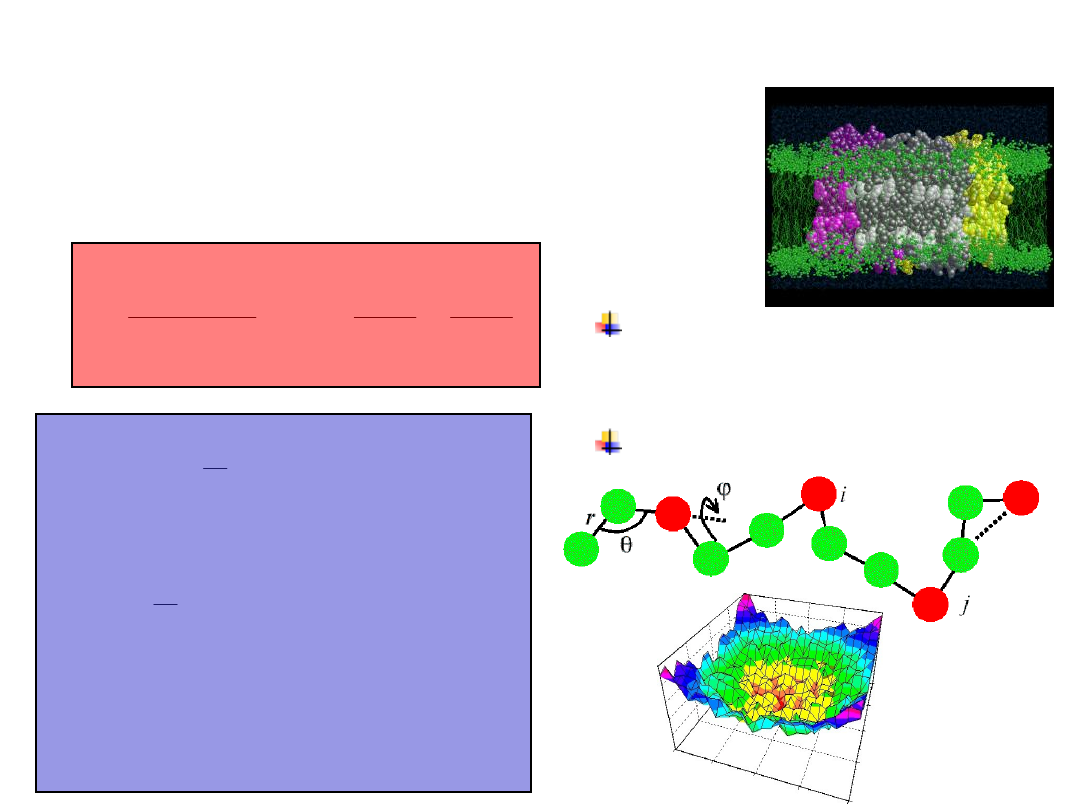There are two types of
interactions

)))

(

cos(

1

(

2

1

2

1

4

0

2

0

,

,

,

,

,

,

2

0

,

,

,

6

,

,

12

,

,

,

0







n

k

k

b

r

k

r

B

r

A

r

q

q

V

dihedrals

angels

k

j

i

k

j

i

k

j

i

bonds

j

i

j

i

b

j

i

j

i

j

i

j

i

j

i

j

i

j

i

j

i

j

i

noncovalent

covalent

Structure of macromolecules and aggregates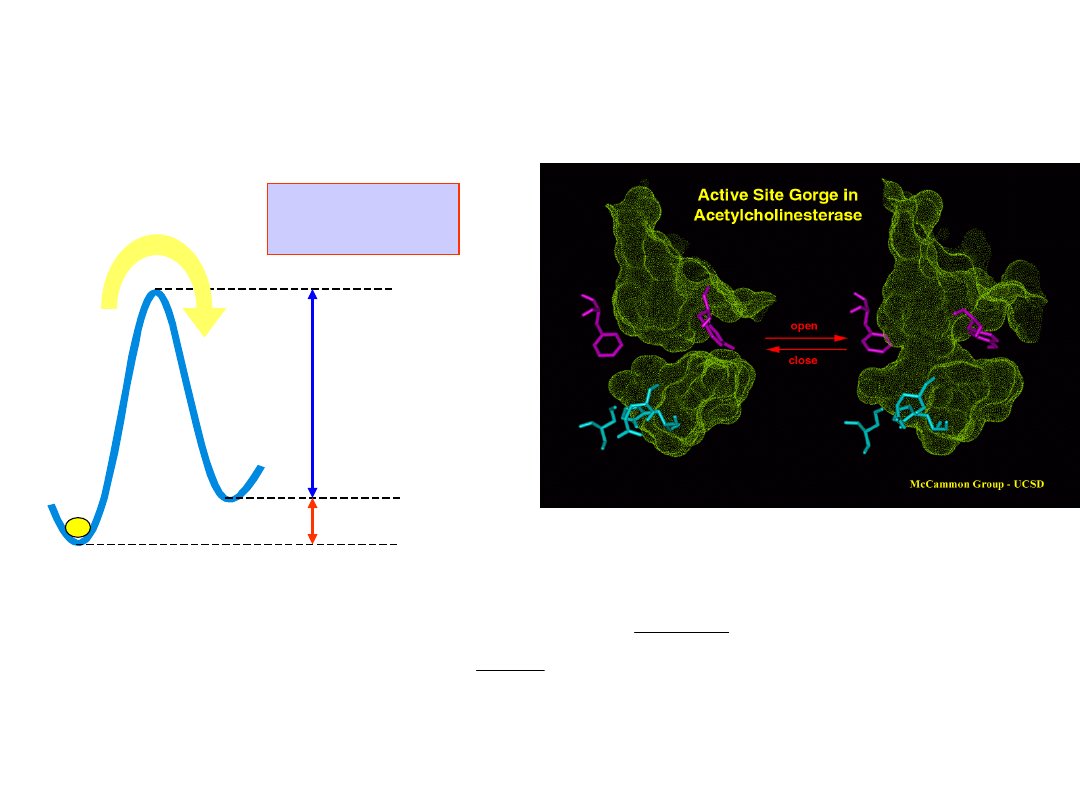Thermal motion

- biomolecules are stable

enough to make things work, and yet allow

the systems to play around in order to allow

the evolution

B

A

o

AB

G

E

a

A

B

T

k

G

B

A

B

o

B

A

e

A

B

K

]

[

]

[

At equilibrium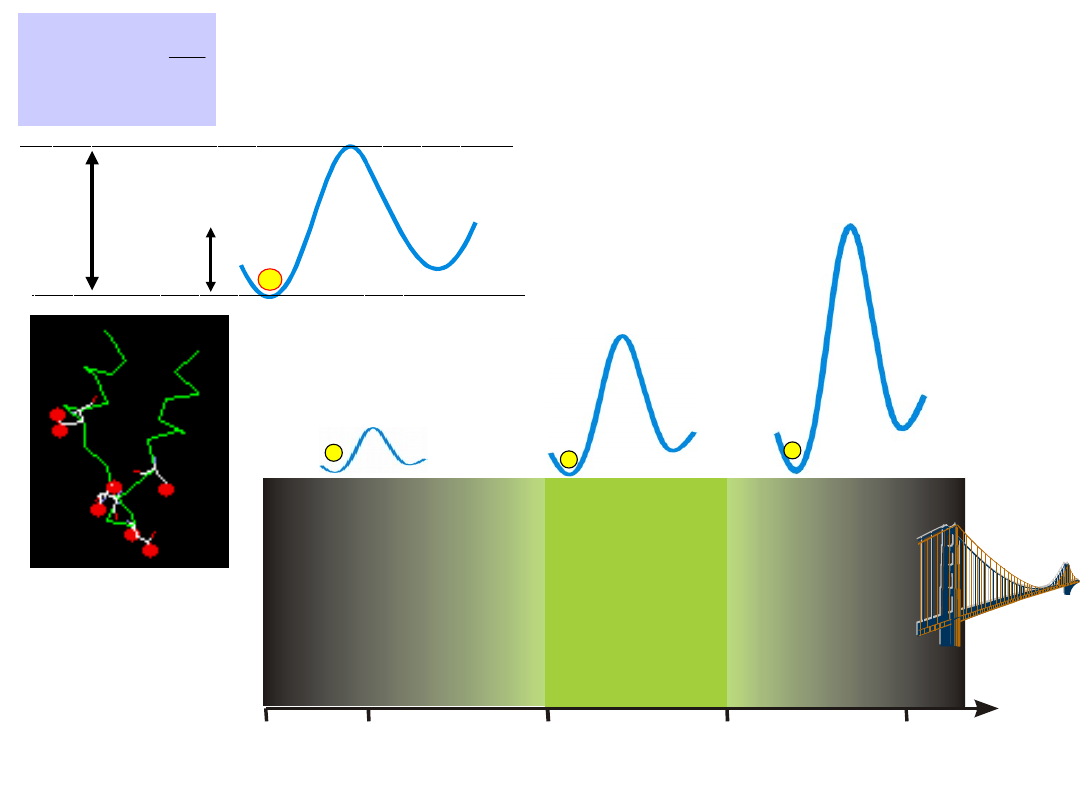0

3 k T

3 5 k T

3

k T

3 0 0 k T

E =

How long it takes for

Brownian motion to

overcome an energy

barrier ?

Brownian death

pico-seconds

Biology

seconds

Too strong

10

67

years

E

a

kT

kT

E

a

e

t

t

0

t

0

~ 10

-12

seconds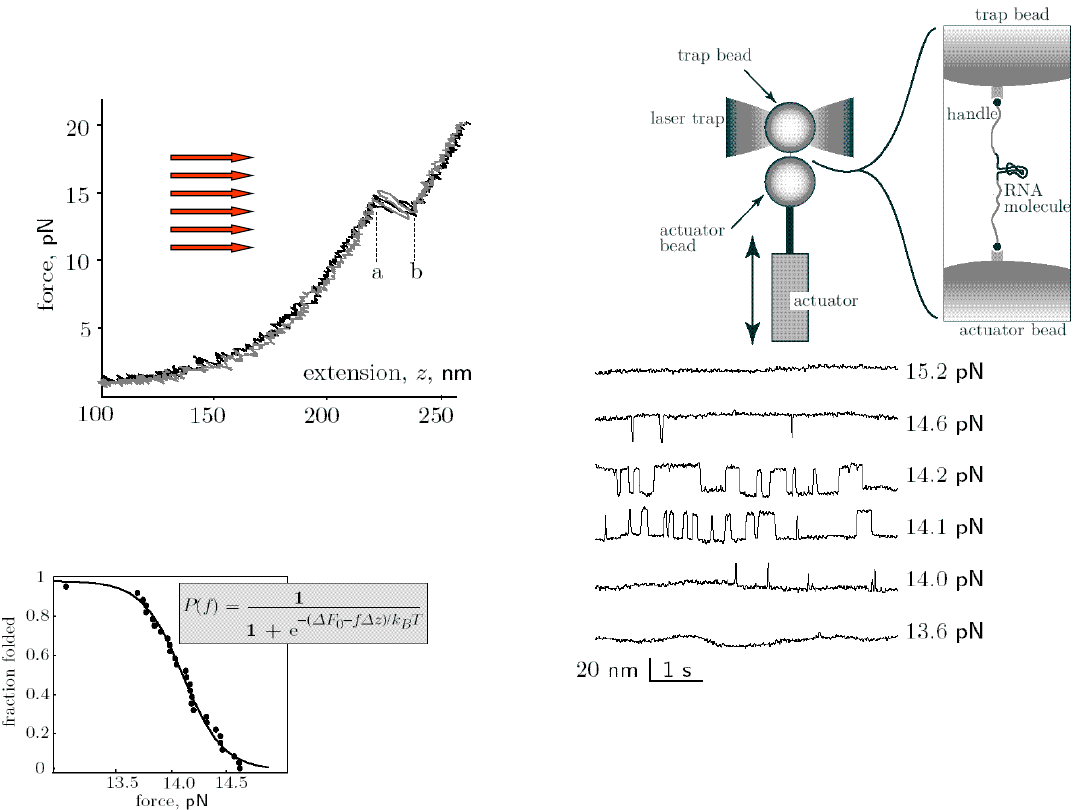Hairpin unfolding

accurs at 14.5 pN

(a)

Force-extension curves of an
RNA hairpin. Scretching and
relaxing

curves

are

superimposed.

DNA folding as a two-state system

Effect of mechnical forcen on the
rate of RNA folding. Length
versus time traces of the RNA
hairpin

at

various

constant

forces.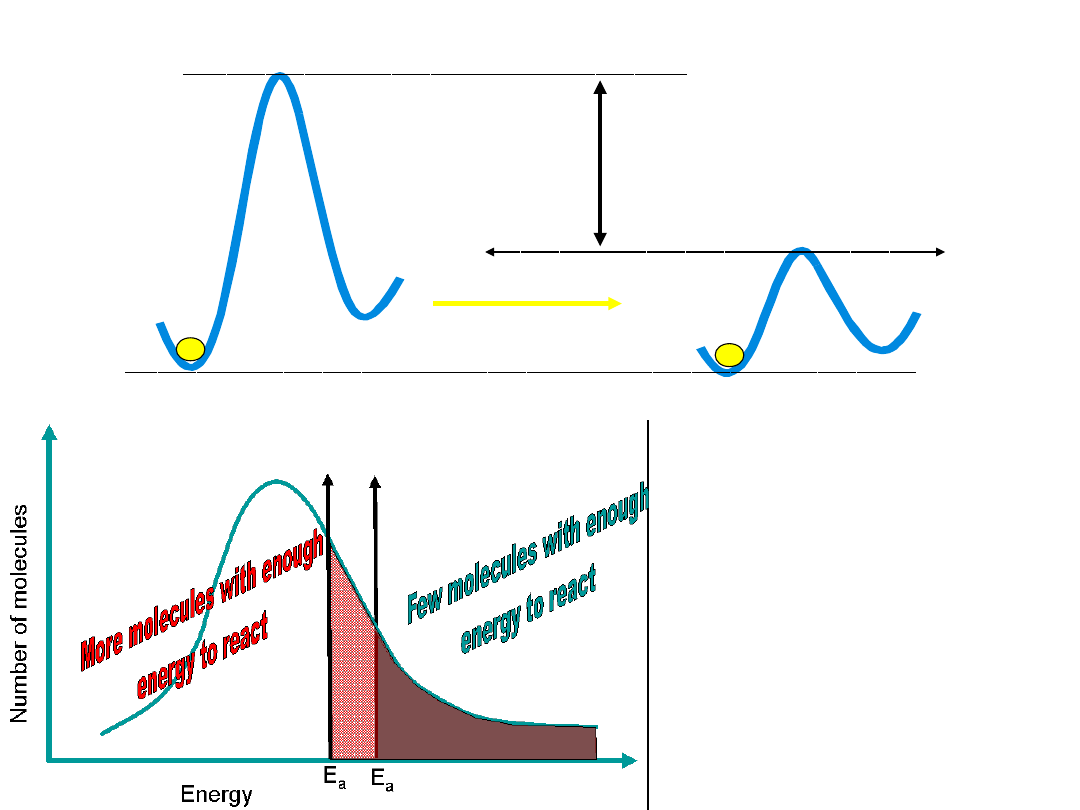E

Control in Biology is accomplished by reducing

energy barriers.

Statistics

Unlikely events

will occur over

short time

periods.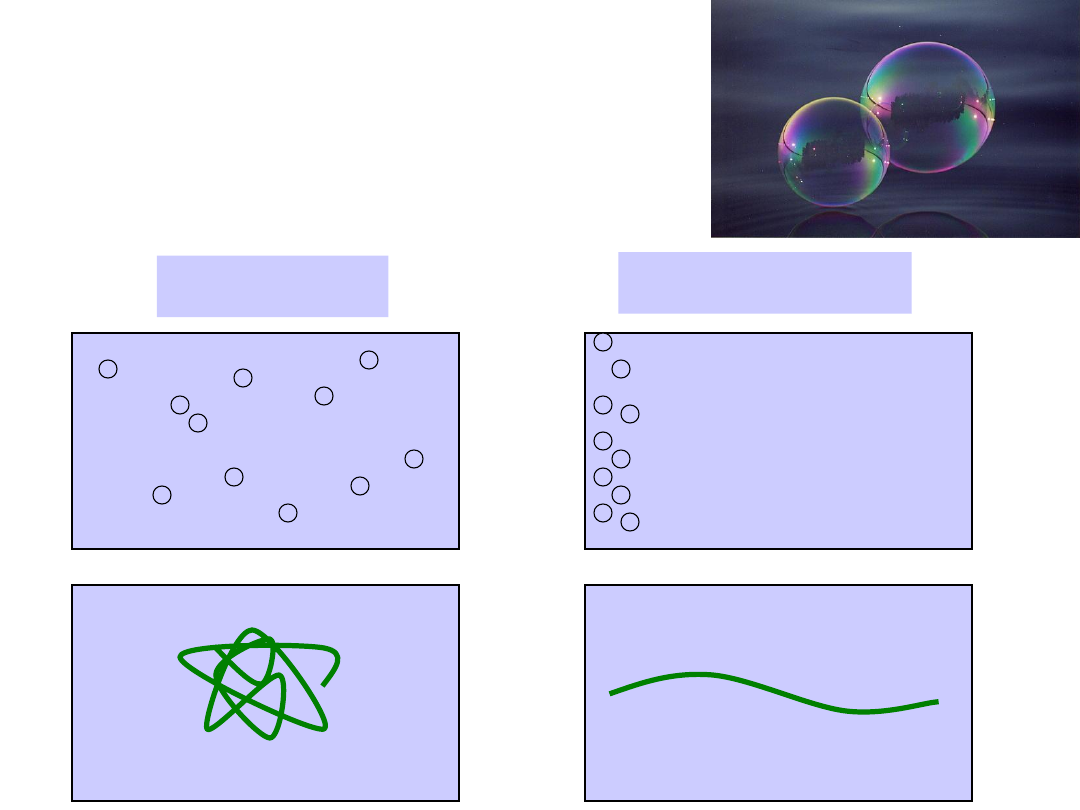Probable

Improbable

Disorder is Favorable

Disorder is Favorable

Entropic

Forces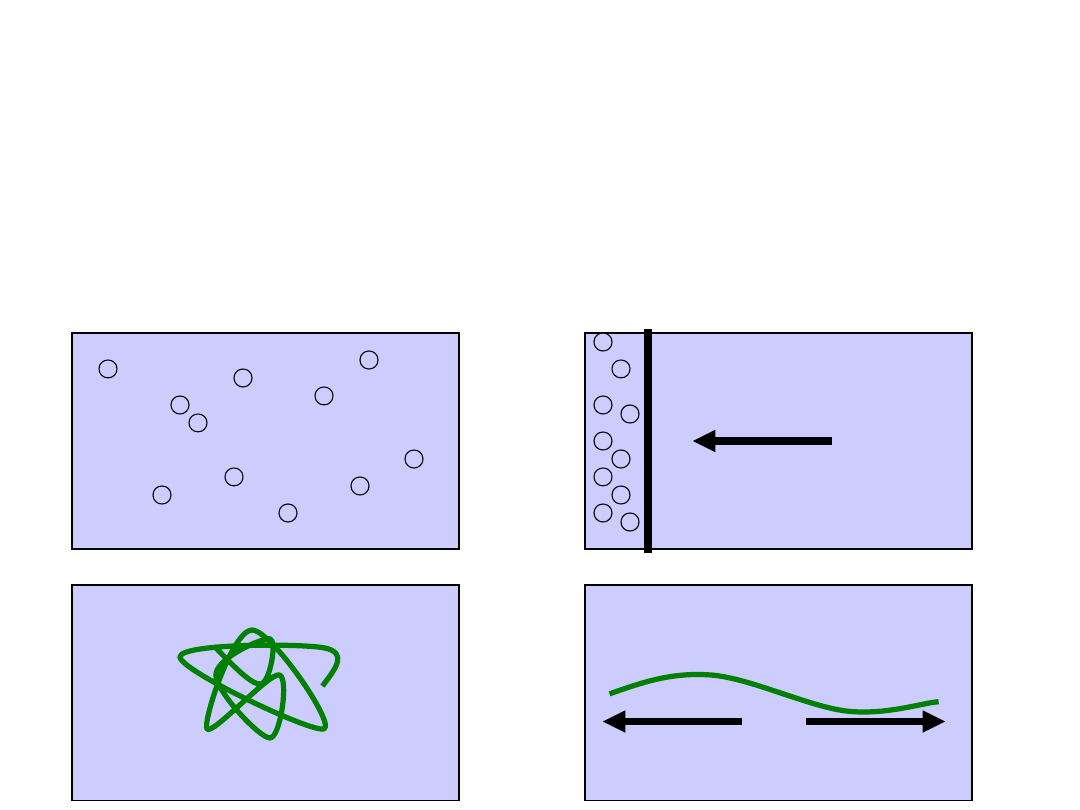Pressure

Tension

To create order

To create order

‘work’ must be

‘work’ must be

done

done

The entropic forces can create a

situation where two molecules will

interact strongly, although there is

not a direct “force” between them.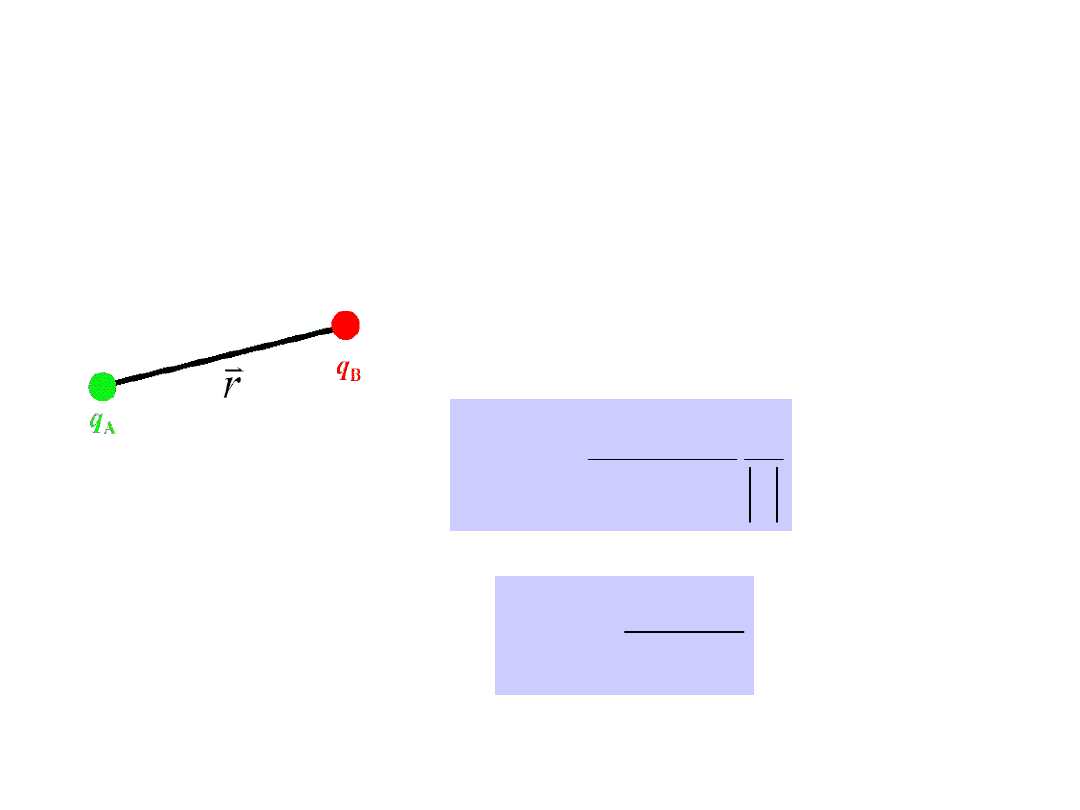Electrostatics

Charge-charge interaction

r

q

q

r

U

B

A

0

4

)

(



r

r

r

q

q

r

F

B

A

2

0

4

)

(

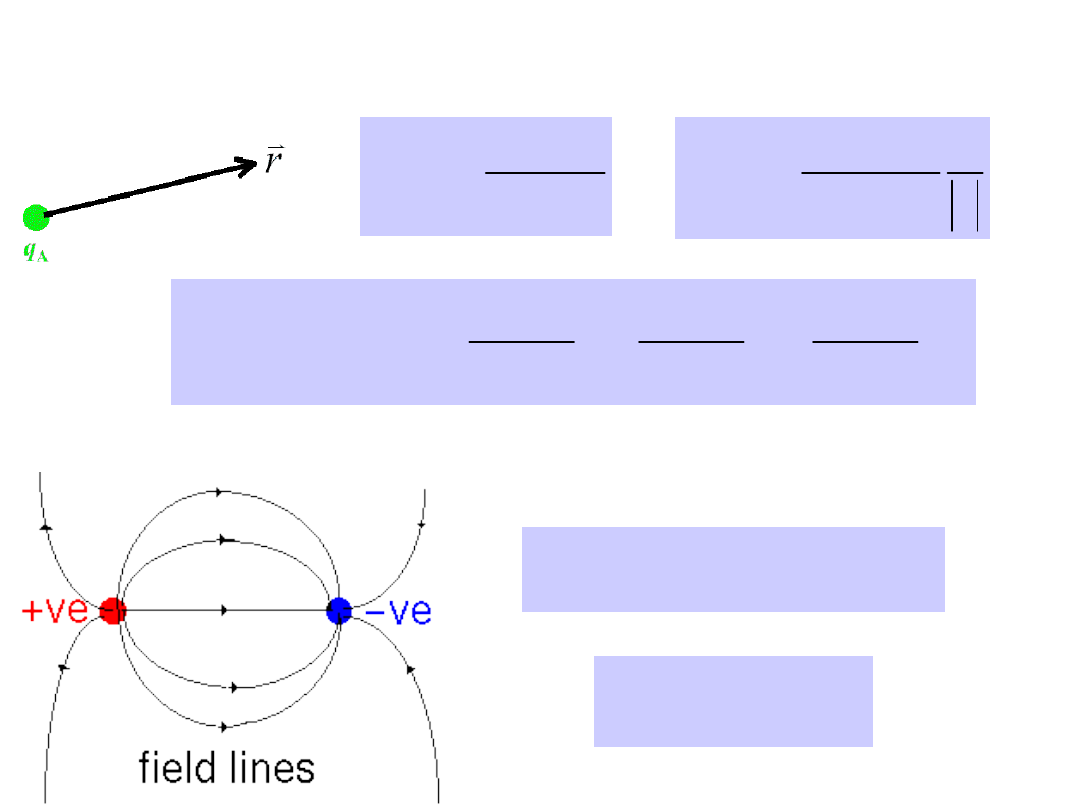Electric field of point charges

r

q

r

V

A

0

4

)

(



r

r

r

q

r

E

A

2

0

4

)

(



E

q

r

F

B

)

(

)

(r

V

q

Energy

B







k

dz

r

V

j

dy

r

V

i

dx

r

V

r

V

E

)

(

)

(

)

(

)

(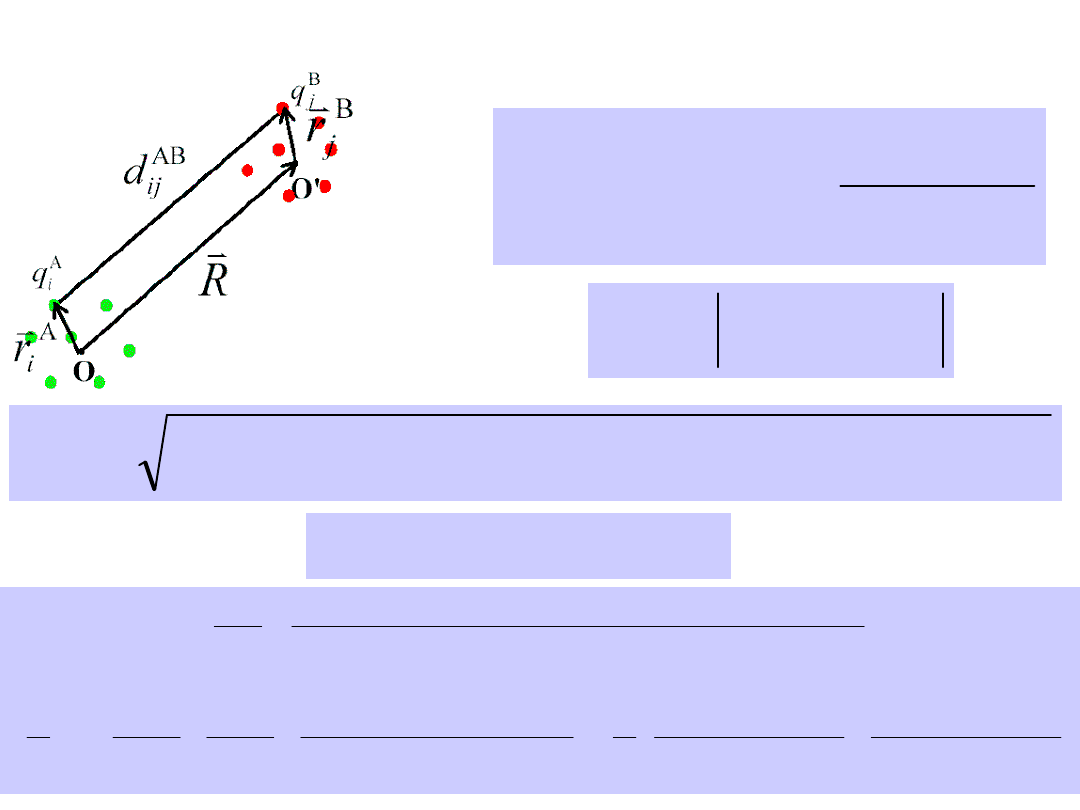Interaction between two charge

distributions

j

i

j

i

AB

ij

B

j

A

i

AB

ij

d

q

q

U

U

,

,

0

4



A

i

B

j

AB

ij

r

r

R

d

B

j

A

j

A

j

B

j

A

j

B

j

AB

ij

r

r

r

R

r

R

r

r

R

d

2

2

2

)

(

)

(

2

2

2

R

r

and

R

r

B

i

A

i







 

 

2

2

2

2

2

2

2

2

/

1

2

2

2

2

3

2

3

1

3

1

1

2

2

2

)

(

)

(

1

1

R

r

r

R

R

r

r

R

R

R

r

R

r

R

r

r

R

r

R

R

r

R

R

r

r

r

R

r

R

r

r

R

d

B

j

B

i

A

j

A

i

B

i

A

i

B

j

A

i

B

i

A

i

B

j

A

j

A

j

B

j

A

j

B

j

AB

ij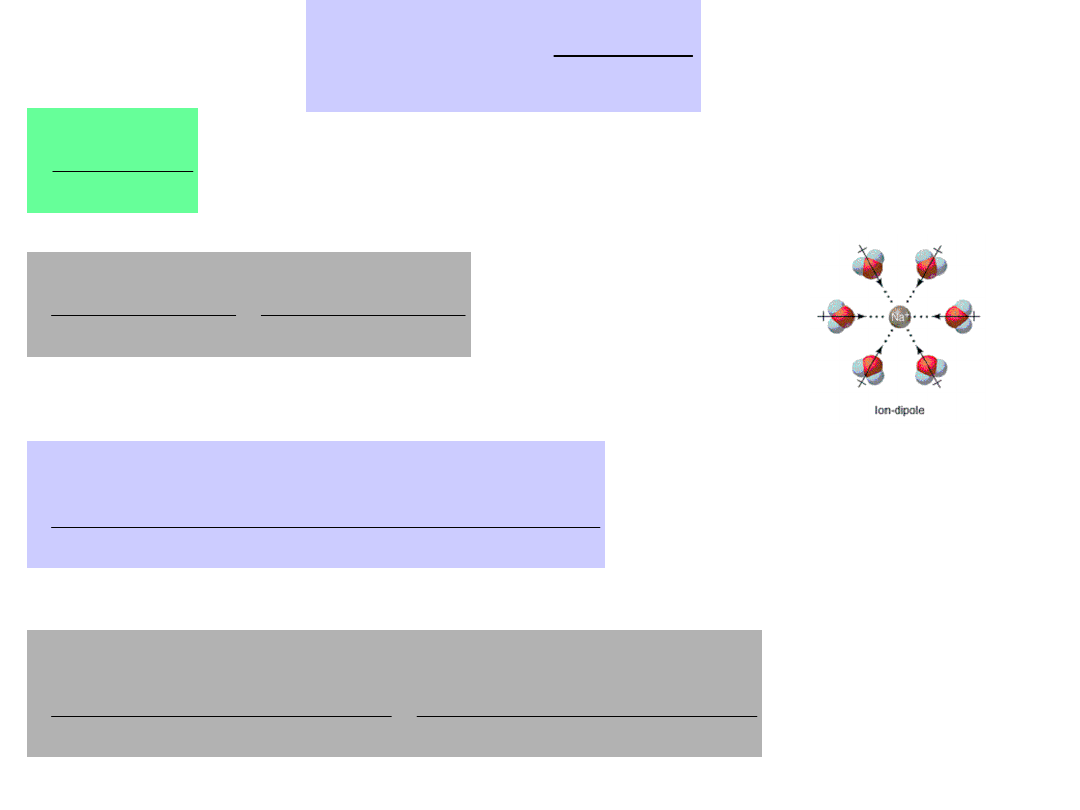j

i

j

i

AB

ij

B

j

A

i

AB

ij

d

q

q

U

U

,

,

0

4



 

 

3

0

2

2

3

0

2

2

8

3

8

3

R

r

r

R

q

q

R

r

r

R

q

q

j

B

j

B

j

B

j

i

A

i

i

A

i

A

i

A

i

j

B

j





dipole-dipole

3

0

4

3

R

r

q

R

r

q

R

r

q

r

q

j

B

j

B

j

i

A

i

A

i

i

j

B

j

B

j

A

i

A

i











charge-charge

R

q

q

i

j

B

j

A

i

0

4



 

charge-dipole

2

0

2

0

4

4

R

r

q

R

q

R

r

q

R

q

i

j

B

j

B

j

A

i

j

i

A

i

A

i

B

j



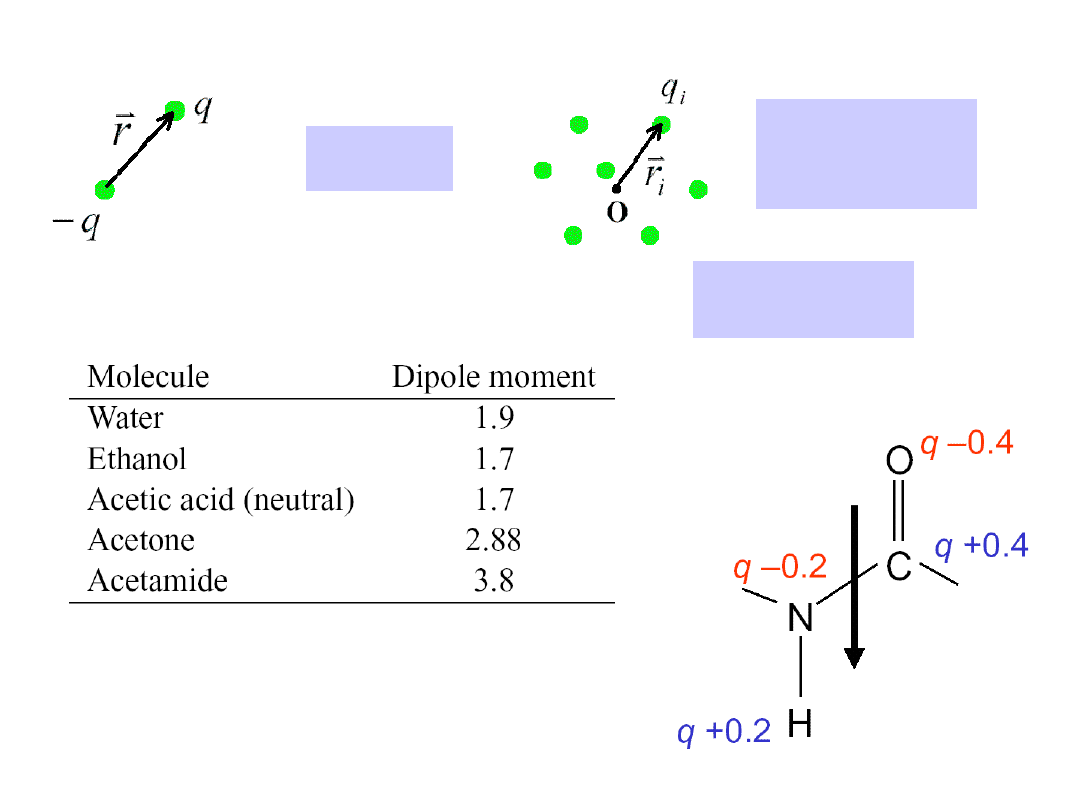i

i

i

r

q

p

Electric dipole
moment

r

q

p

p

p = 3.8 Debya

E

p

U

Interaction energy of a dipole with a field

1 Debye = 0.2 electron-Angstroms
= 3.3x10

–23

Cm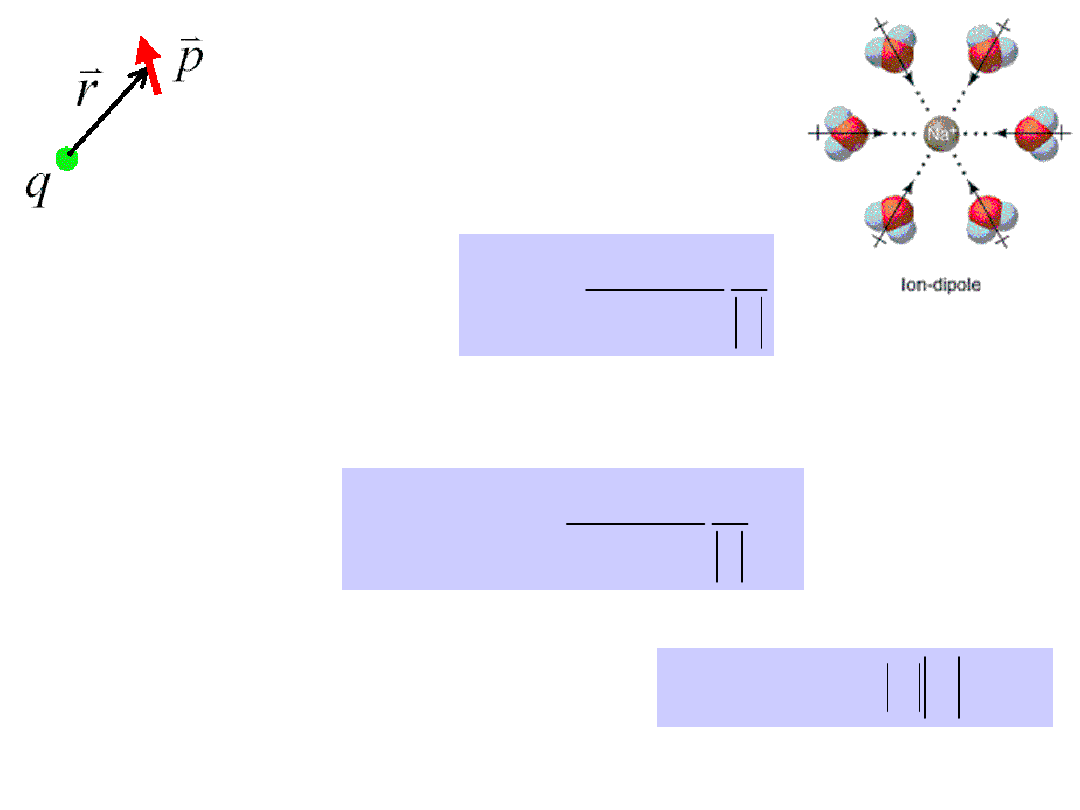Electric field of a
charge

r

r

r

q

r

E

2

0

4

)

(



Interaction energy between a charge and a dipole

p

r

r

r

q

E

p

U

2

0

4



Charge-dipole interaction

The energy of a dipole p in an electric
field

 - the angle between the dipole and the

electric field.

cos

E

p

E

p

U

p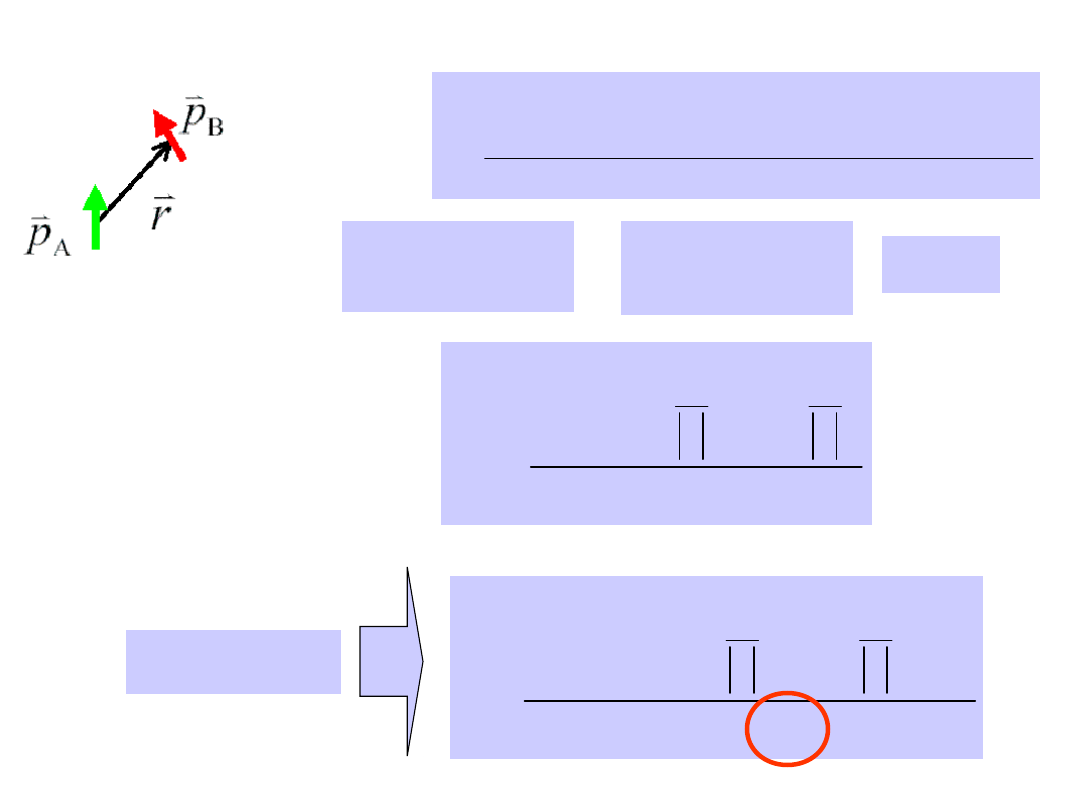i

A

i

A

i

A

r

q

p

j

B

j

B

j

B

r

q

p

r

R

A

B

E

p

U

Electric field of a dipole

3

0

4

3

r

r

r

p

r

r

p

E

A

A

A



3

0

4

3

R

r

q

R

r

q

R

r

q

r

q

U

j

B

j

B

j

i

A

i

A

i

i

j

B

j

B

j

A

i

A

i











Dipole-dipole interaction

3

0

4

3

r

p

r

r

p

r

r

p

p

U

B

A

B

A









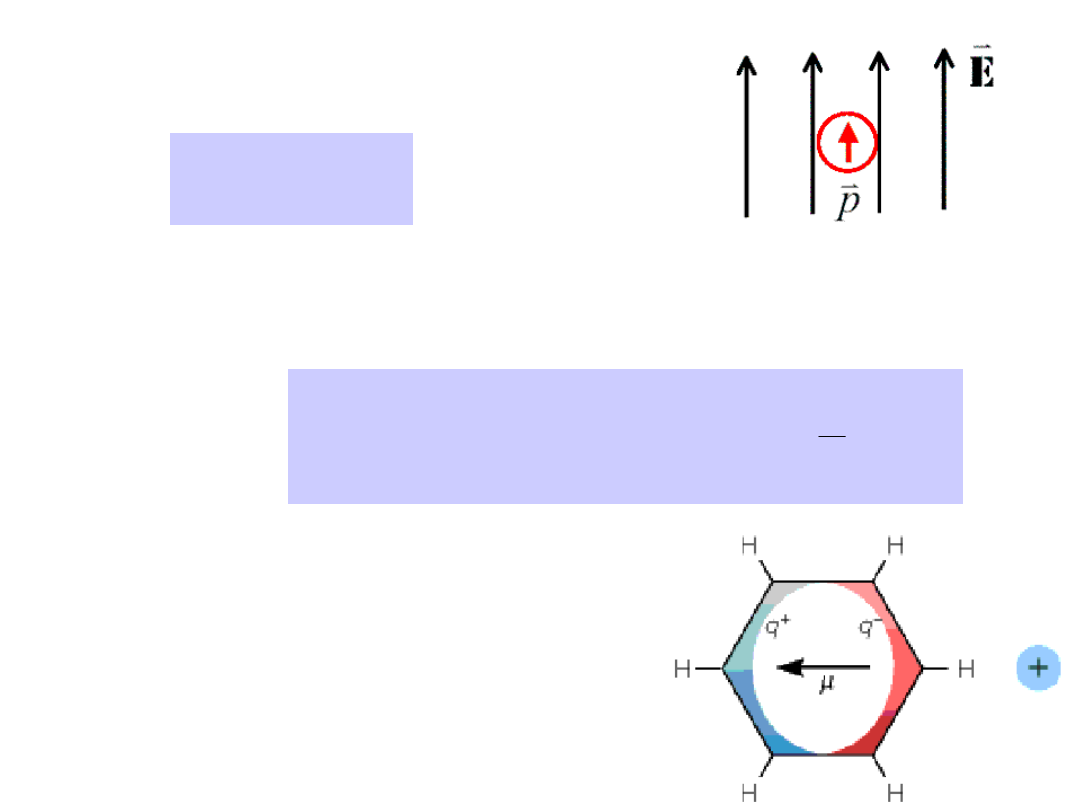A

E

p

0



– polarizability of the molecule

Inducede
dipole

Interaction energy between the induced dipole and the
inducing field

2

0

0

0

0

2

1

E

E

d

E

E

d

p

U

E

E





Induced dipoles: dependent
upon

polarizability

of

molecule, how easily electrons
can shuffle around to react to
a charge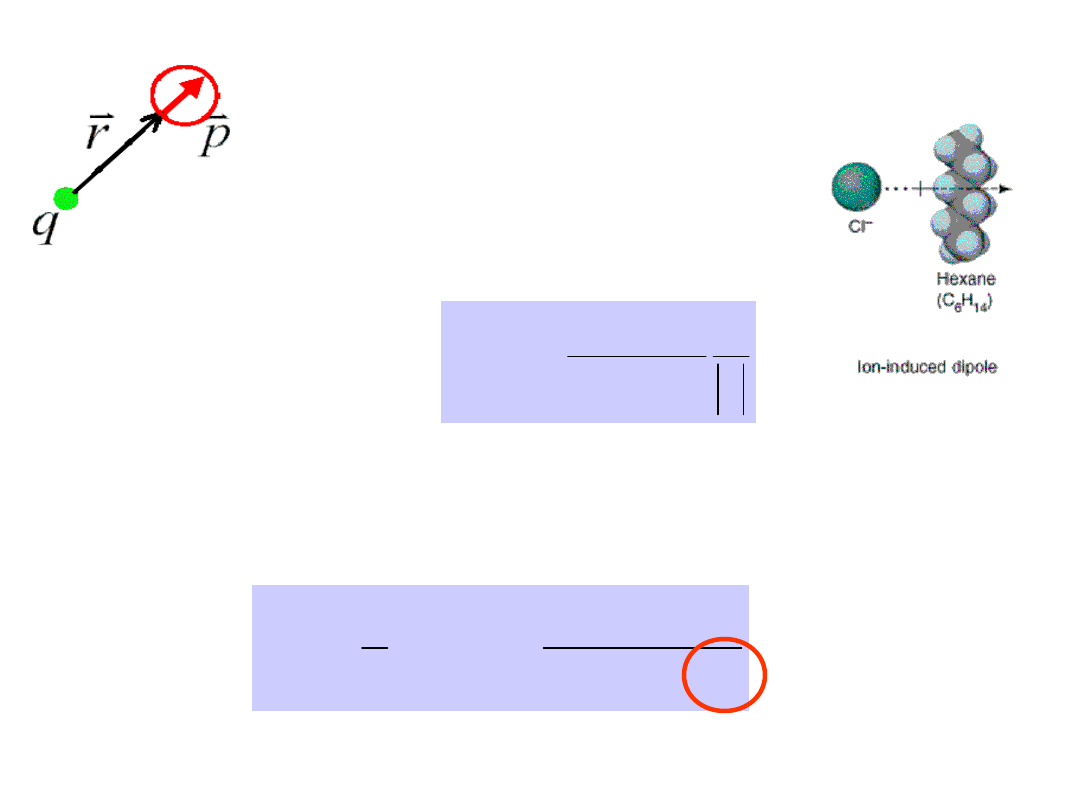Electric field of a
charge

r

r

r

q

r

E

A

2

0

4

)

(



Interaction energy between a charge and its induced dipole

4

0

2

2

2

2

0

32

2

1

r

q

E

U



Charge-induced dipole interaction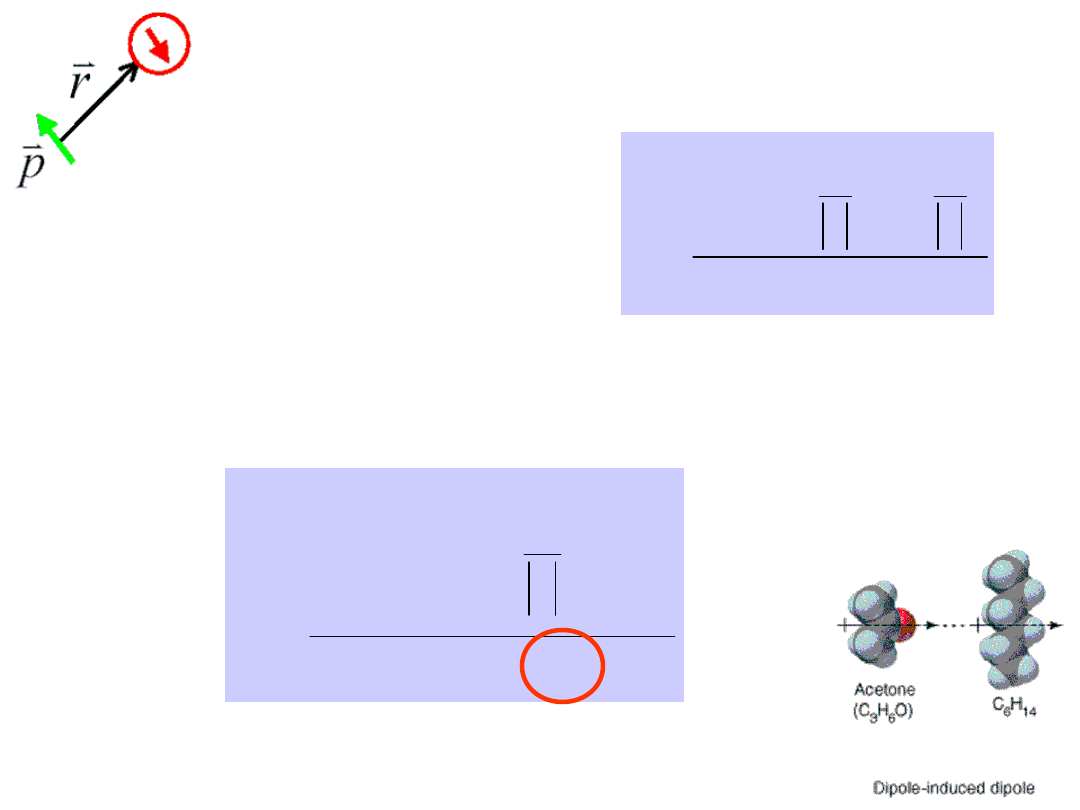Electric field of a dipole

3

0

4

3

r

r

r

p

r

r

p

E



Dipole-induced dipole interaction

Interaction energy between a dipole and its induced dipole

6

0

2

2

2

32

3

r

p

r

r

p

U

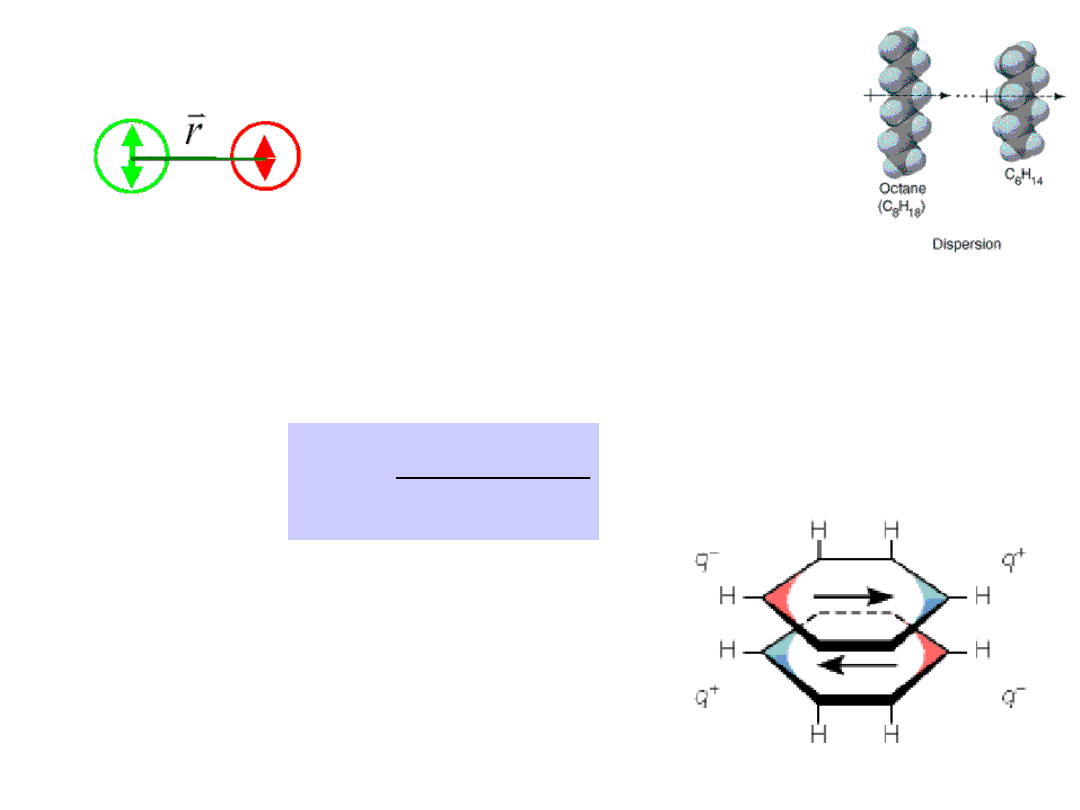Induced dipole-induced dipole interaction

Interaction between two harmonic oscillators with
natural frequency 

1

and 

2

and polarizabilities 

1

and 

2

.

6

2

1

2

1

2

1

)

(

2

3

r

v

v

v

hv

U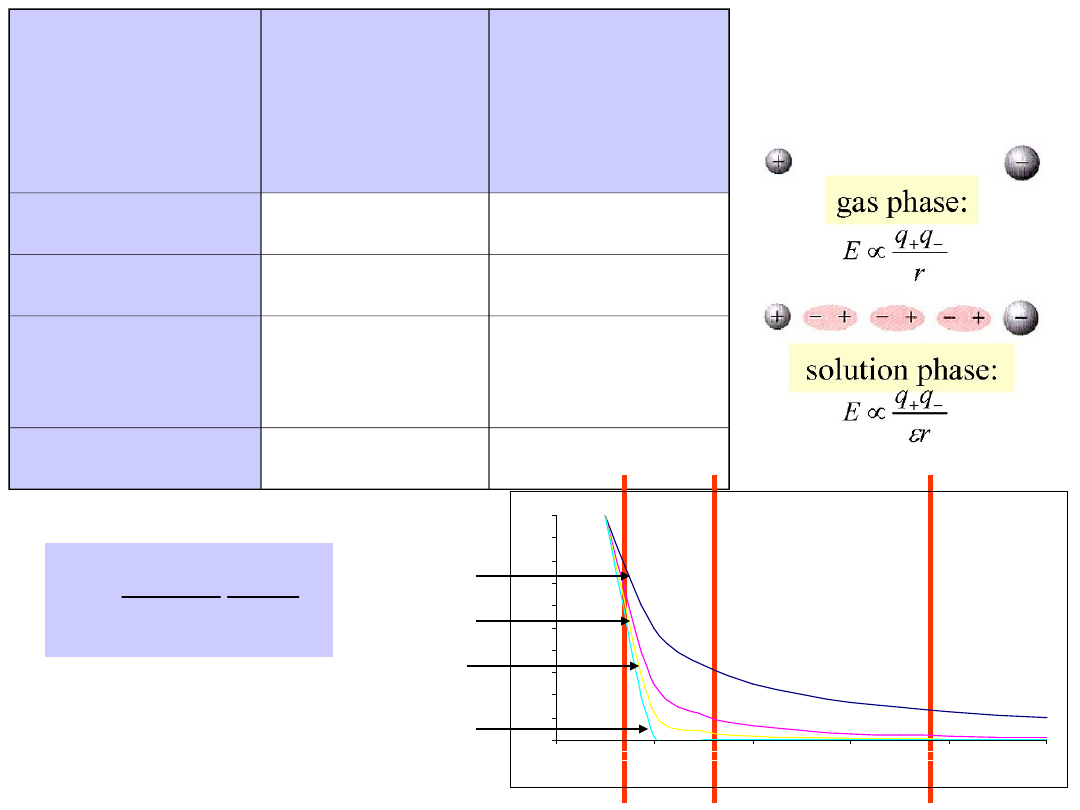Summary

System

Potential

dependen

ce on

distance

Energy

[kJ/mol]

ion-ion

r

–1

250

ion-dipol

r

–2

15

dipol –

dipol

r

–3

2

London

r

–6

1

0

0,1

0,2

0,3

0,4

0,5

0,6

0,7

0,8

0,9

1

0

2

4

6

8

10

r

-1

r

-2

r

-3

r

-6

Water Screens

Electrostatic

Forces

r

r

q

q

F

3

2

1

0

4

1





~ 1-10 for organic substances



= 80 for pure water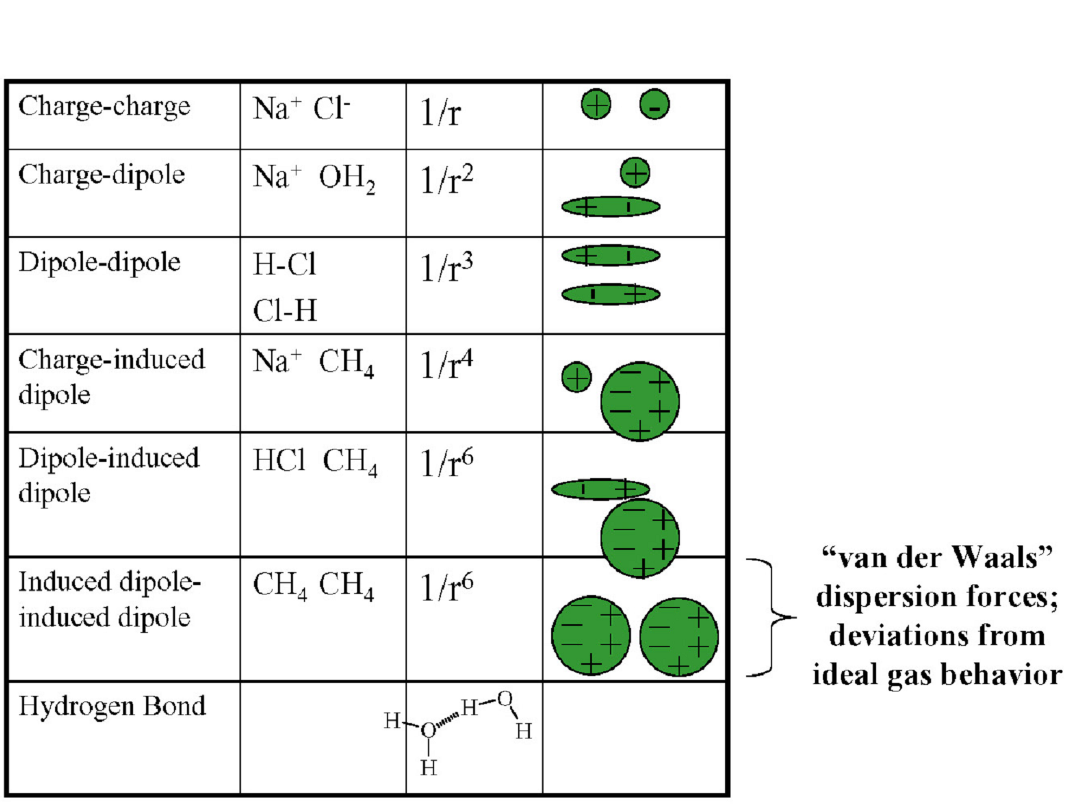Summary

# Document Outline

Wyszukiwarka

Podobne podstrony:
Gwinty, wyklad 04 polaczenia srubowe CRC A717D1E6
Prawo konkurencji wykład 7 - 04.12, WPiA UŁ, Prawo ochrony konkurencji i konsumentów (T. Ławicki)
Młoda Polska WYKŁAD (04 06 2014)
Podstawy Systemów Okrętowych wykład 04 Przeciw Pożarnicze